[BACK]Computer Modeling in Engineering & SciencesDOI: 10.32604/cmes.2021.016803

ARTICLE

Mass-Stiffness Templates for Cubic Structural Elements

Department of Aerospace Engineering Sciences and Aerospace Mechanics Research Center, University of Colorado at Boulder, Boulder, CO 80309-0429, USA
*Corresponding Author: Carlos A. Felippa. Email: carlos.felippa@colorado.edu
Received: 26 April 2021; Accepted: 06 July 2021

Abstract: This paper considers Lagrangian finite elements for structural dynamics constructed with cubic displacement shape functions. The method of templates is used to investigate the construction of accurate mass-stiffness pairs. This method introduces free parameters that can be adjusted to customize elements according to accuracy and rank-sufficiency criteria. One- and two-dimensional Lagrangian cubic elements with only translational degrees of freedom (DOF) carry two additional nodes on each side, herein called side nodes or SN. Although usually placed at the third-points, the SN location may be adjusted within geometric limits. The adjustment effect is studied in detail using symbolic computations for a bar element. The best SN location is taken to be that producing accurate approximation to the lowest natural frequencies of the continuum model. Optimality is investigated through Fourier analysis of the propagation of plane waves over a regular infinite lattice of bar elements. Focus is placed on the acoustic branch of the frequency-vs.-wavenumber dispersion diagram. It is found that dispersion results using the fully integrated consistent mass matrix (CMM) are independent of the SN location whereas its low-frequency accuracy order is O(κ8), where κ is the dimensionless wave number. For the diagonally lumped mass matrix (DLMM) constructed through the HRZ scheme, two optimal SN locations are identified, both away from third-points and of accuracy order O(κ8). That with the smallest error coefficient corresponds to the Lobatto 4-point integration rule. A special linear combination of CMM and DLMM with nodes at the Lobatto points yields an accuracy of O(κ10) without any increase in the computational effort over CMM. The effect of reduced integration (RI) on both mass and stiffness matrices is also studied. It is shown that singular mass matrices can be constructed with 2-and 3-point RI rules that display the same optimal accuracy of the exactly integrated case, at the cost of introducing spurious modes. The optimal SN location in two-dimensional, bicubic, isoparametric plane stress quadrilateral elements is briefly investigated by numerical experiments. The frequency accuracy of flexural modes is found to be fairly insensitive to that position, whereas for bar-like modes it agrees with the one-dimensional results.

Keywords: Structural dynamics; Lagrangian elements; finite elements; cubic shape functions; bar; plane stress; mass; stiffness; vibration; wave propagation; Fourier analysis; dispersion; templates

1  Introduction

The accompanying contribution in this issue  narrates the author’s effort in understanding and draining the “variational swamp” that emerged during 1965-1985. In those two decades the Finite Element Method (FEM) was liberated from the Rayleigh-Ritz connection, but fragmented developments had resulted in a dizzying array of variational tools. The effort resulted in the unification published in , which made a wide range of classical principles in structural mechanics (e.g., Total Potential Energy, Hellinger-Reissner, Veubeke-Hu-Washizu, etc.) particular cases of a single parametrized functional. Setting parameters to specific values produced well known instances, plus an infinity of new ones.

A practical side effect: element derivations could be carried out by splitting the formulation and using two or more functionals. The split could be geometric: element boundary plus interior, as in the by-now large class of hybrids and incompatible models . Or additive: basic plus higher order response, as in Bergan’s Free Formulation . Or splitting done in the matrix computation process, as in elements produced by selective integration .

Investigation of common features emerging in those combinations led to the method of templates, which provides tools for the present paper. Templates are algebraic forms for the mass, material and geometric stiffness matrices of an individual element. Distinguishing feature: templates contain free parameters. These may be either simply scaling coefficients, or carry immediate physical meaning. In this paper two parameters are used:

•   A geometric parameter γ that adjusts the side node location.

•   A scaling parameter μ that defines the combination of consistent and diagonally-lumped mass matrices.

A short history of templates, adapted from a recent survey paper  is given in Appendix A. The present paper is a specialized continuation of a 2015 survey paper  that studies mass-stiffness templates for structural dynamics in a more general context, following up on earlier work [10,11].

2  Lagrangian Cubic Elements

Lagrangian structural elements constructed with cubic displacement shape functions possess only translational degrees of freedom (DOF). They carry additional nodes in addition to corners, Fig. 1. These will be denoted as “side nodes” (SN) in the sequel, although for one-dimensional (1D) elements such as that shown in Fig. 1a, they are also internal nodes. SN are usually placed at the thirdpoints, but their location may be symmetrically adjusted, within geometric limits, using a template parameter. For multidimensional Lagrangian elements such as those pictured in Figs. 1c1e, the SN location determines placement of internal and face nodes.

The SN adjustment effect is studied here in detail for the simplest case: the four-node, elastic, prismatic, cubic bar element of Fig. 1a. The Mathematica computer algebra system is used for symbolic computations. Key results are then verified with numerical calculations. The stiffness and mass matrices are constructed through isoparametric cubic shape functions parametrized as per SN location.

The approximation properties are determined by Fourier analysis of plane waves propagating over an infinite lattice of identical elements. This provides dispersion diagrams that connect natural frequencies to dimensionless wavenumbers. Those diagrams include one acoustical branch and two optical branches. Of these the acoustic branch is of paramount interest as regards approximation of continuum natural frequencies. On the other hand, optical branches are physically spurious and a byproduct of the discretization process (They affect primarily the so-called numerical pollution).

The result of changing the SN location on acoustic branch accuracy is further studied for several mass-stiffness combinations differing on Gauss integration rules. The effect of mass lumping, as well as taking a linear combination of the consistent and diagonaly-lumped matrices, is considered.

The paper concludes with a numerical investigation of the performance of 2D plane stress Lagrangian elements in a beam vibration problem. Their inherent complexity makes symbolic analysis unfeasable on present computer algebra systems, a limitation explained in Appendix A.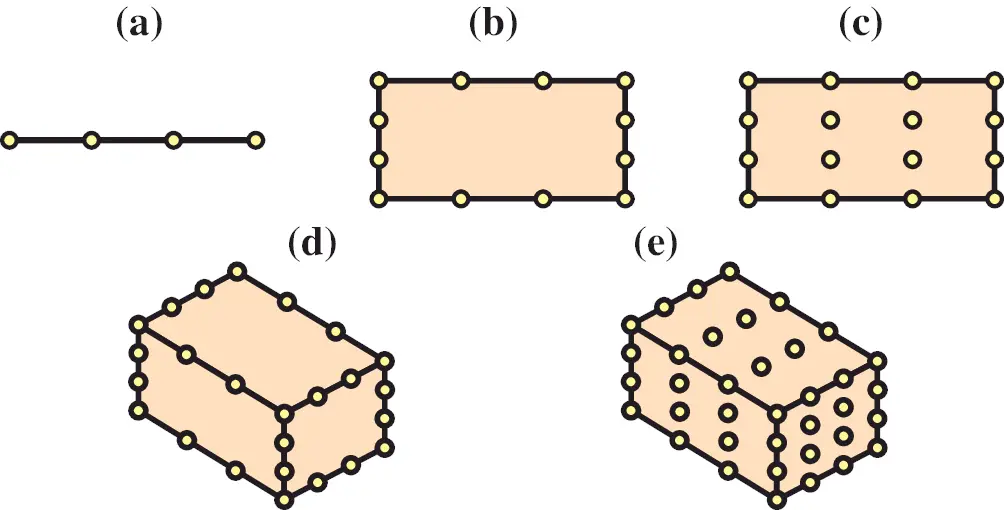Figure 1: Cubic elements with side nodes: (a) 4-node bar; (b) 12-node serendipity quadrilateral; (c) 16-node Lagrangian quadrilateral; (d) 32-node serendipity brick; (e) 64-node Lagrangian brick. Non-corner nodes drawn at the thirdpoints for convenience. For (d, e) only visible nodes are shown

3  The Four-Node Bar (Bar4) Element

Consider a four-node prismatic bar element of length l, constant mass density ρ, elastic modulus E, and cross section area A, which can only move along its longitudinal axis, Fig. 2. This model is acronymed Bar4 for brevity’s sake in the sequel. Nodes are locally numbered as shown. The axial coordinate is x with origin at element center. The axial displacement is u = u(x). The isoparametric (isoP) natural coordinate ξ varies from ξ = −1 to ξ = 1 from end Node 1 to end Node 2, respectively. The position of the side nodes (SN) is specified by the template parameter γ shown in the figure, so that their isoP coordinates are ξ = ±γ. The SN arrangement is constrained to be symmetric respect to the center ξ = 0. If the sign of γ is reversed, the nodes just flip, whence the allowable position range is taken to be γ ∈ (0, 1), which excludes γ = 0 and γ = 1.

3.1 Bar4 Shape Functions

The isoparametric formulation based on the γ-parametrized shape functions

N1e=12(1ξ)(ξ2γ2)1γ2,N2e=12(1+ξ)(ξ2γ2)1γ2,N3e=12(1ξ2)(ξγ)γ(1γ2),N4e=12(1ξ2)(ξ+γ)γ(1γ2), (1)

is used. For γ = 1/3, (1) are the well known cubic shape functions for equidistant nodes . If γ=1/50.4472135955 they become the 4-point Lobatto shape functions. The shape function matrix is

Ne=[N1eN2eN3eN4e]. (2)

The overall element isoparametric definition can be concisely written 

[xu]=[N1eN2eN3eN4e][x1u1x2u2x3u3x4u4]=[NexNeu]. (3)Figure 2: Configuration of the four-node bar (Bar4) element, showing local node numbering as well as geometric, constitutive and fabrication properties. Side Nodes 3–4 are symmetrically placed about the center, at distances ±γl/2

Here both x and u are column 4-vectors, with x=12[11γγ]T. Expanding: x=Nex=(N1e+N2eγN3e+γN4e)=ξ, whence the Jacobian determinant is

J=dxdξ=12. (4)

This is constant over the element and independent of γ, which greatly simplifies integration.

3.2 Bar4 Strain-Displacement Equations

The natural derivatives of (1) are

N1,ξe=dN1edξ=γ2+2ξ3ξ22(1γ2),N2,ξe=dN2edξ=γ2+2ξ+3ξ22(1γ2),N3,ξe=dN3edξ=12γξ+3ξ22γ(1γ2),N4,ξe=dN4edξ=12γξ3ξ22γ(1γ2). (5)

The axial strain is given by

e=dudx=dudξdξdx=J1dudξ=2dudξ=def[B1eB2eB3eB4e][u1u2u3u4]=Beu. (6)

Here Be is the 1 × 4 strain-displacement matrix with entries Bie=J1dNie/dξ for i = 1,…4.

4  Bar4 Mass and Stiffness Matrices

The element consistent mass matrix (CMM), parametrized in terms of γ, is defined as

MCe(γ)=/2/2ρA(Ne)TNedx=11ρA(Ne)TNeJdξ, (7)

Here Ne is the 1 × 4 shape function matrix (3), which depends on γ. For a prismatic homogeneous element, ρ and A may be taken out of the integral, as well as J = l/2. The expression (7) can be evaluated using Gauss integration rules with pM points, in which case pM is appended as second argument of MCe. For constant ρ, A and J:

MCe(γ,pM)=ρAJk=1pMwk(Nke)TNke,withNke=Ne|ξξk, (8)

in which wk and ξk are weights and abcissas, respectively, of the pM-point Gauss rule. Since the entries of Ne are cubic polynomials in ξ, exactness is attained if pM4.

The element stiffness matrix, parametrized in terms of γ, is given by

KCe(δ)=/2/2EA(Be)TBedx=11EA(Be)TBeJdξ, (9)

in which Be is the γ-dependent strain-displacement matrix (6). For a prismatic homogeneous element, E and A may be taken out of the integral, as well as J = l/2. The expression (9) can be evaluated by a Gauss rule with pK points, in which case pK is appended as second argument of Ke:

KCe(γ,pK)=EAJk=1pKwk(Bke)TBke,withBke=Be|ξξk. (10)

The entries of Be are quadratic polynomials in ξ, whence exactness is attained if pK3.

4.1 Fully Integrated Mass Matrices

The fully integrated (FI) consistent mass matrix (CMM) is obtained by evaluating the integral (7) either analytically, or through the Gauss rule (8) with pM = 4. The resulting matrix is rank sufficient. This exact CMM is denoted by

MCe(γ,4)=[MC11MC12MC13MC14MC22MC23MC24MC33MC34symmetricMC44]=ρA[χC11χC12χC13χC14χC22χC23χC24χC33χC34symmetricχC44] (11)

Here MCij and χCij denote physical and dimensionless entries, respectively. The entry dependence on γ is omitted from (11) to reduce clutter. Their expressions may be found in Table 1.

The associated diagonally-lumped mass matrix (DLMM) is denoted by

The diagonal entries are obtained via the HRZ scheme  as the row sums χL1 = χC11 + χC12 + χC13 + χC14, χL2 = χC21 + χC22 + χC23 + χC24, χL3 = χC31 + χC32 + χC33 + χC34, and χL4 = χC41 + χC42 + χC43 + χC44. The entry dependence on γ is omitted from (12) to reduce clutter. Their expressions are listed in Table 1.

4.2 Fully Integrated Stiffness Matrix

The fully integrated (FI) stiffness matrix is obtained by evaluating the integral (9) either analytically, or through the Gauss rule (10) with pK = 3. It will be denoted as

Ke(γ,3)=[K11K12K13K14K22K23K24K33K34symmetricK44]=EA[ψ11ψ12ψ13ψ14ψ22ψ23ψ24ψ33ψ34symmetricψ44] (13)

Here Kij and ψij denote physical and dimensionless entries, respectively. To reduce clutter their dependence on γ is omitted. Their expressions may be found in Table 2. This matrix has the full rank of 3. Its only zero-energy mode is the rigid body translation along x.

4.3 Reduced Integration Mass and Stiffness Matrices

There has been recent work on nonstandard methods for constructing mass matrices, e.g., . In particular, singular element mass matrices have attracted attention for direct time integration (DTI) computations, as a scheme to reduce numerical pollution caused by spurious optical branches [18,19] This is important in problems exhibiting rapid transients, as well as in multibody dynamics. Three procedures for generating singular mass matrices are described in the survey paper :

•   Spectrally truncated templates

•   Projection onto a null subspace

•   Reduced integration (RI)

Of these, only RI will be considered here because it links up smoothly with the fully integrated (FI) case. RI mass matrices of interest are obtained using Gauss rules with pM = 2 or pM = 3 points. The resulting CMM are denoted by MCe(γ,pM) and likewise for the associated DLMM constructed through the HRZ scheme.

Terminology: an eigenvector corresponding to a zero eigenvalue is called a null eigenvector. If the zero eigenvalue is multiple, the set of all null eigenvectors forms a null eigenspace. Spurious modes are null eigenvectors devoid of physical meaning.

The CMM with pM=3 has rank deficiency 1, which pertains to a spurious cubic mode that vanishes at all three Gauss points. The CMM with pM=2 has rank deficiency 2, which pertains to quadratic and cubic modes that vanish at both Gauss points. Their entries may be found in Table 1. (The pM = 1 version is of no interest.) Associated diagonally lumped matrices do not change.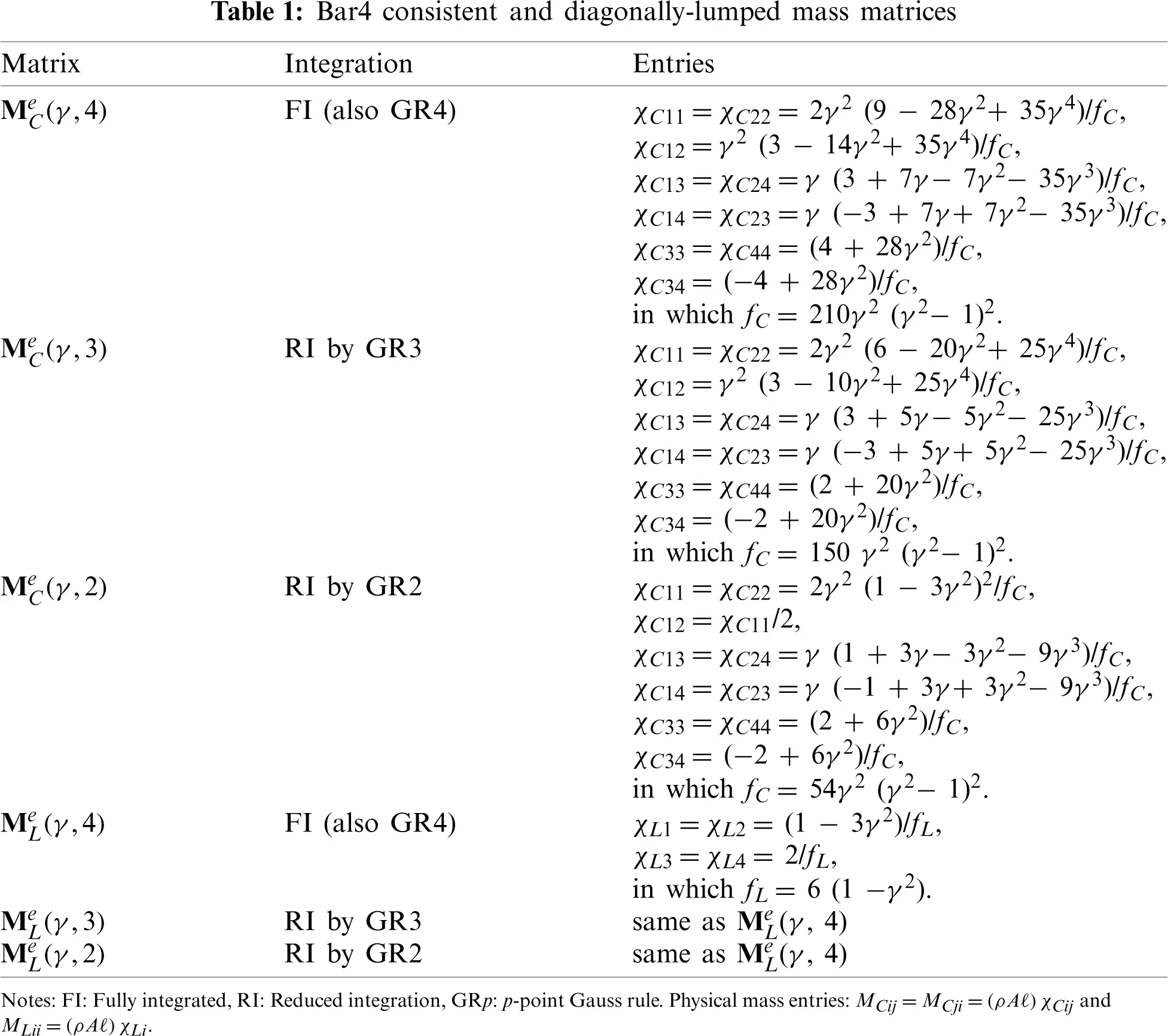A RI stiffness matrix is produced by a Gauss rule with 1 ≤ pK ≤ 2 points, denoted by K(γ, pK). Of the two possibilities, only Ke(γ, 2) computed through the 2-point rule, is of some interest. This matrix has a rank deficiency of 2. The null subspace includes the physical rigid body mode, (uniform longitudinal motion) as well as a cubic mode whose strains vanish at the 2 Gauss points. The matrix is expressed in the form (9). Its entries may be found in Table 1.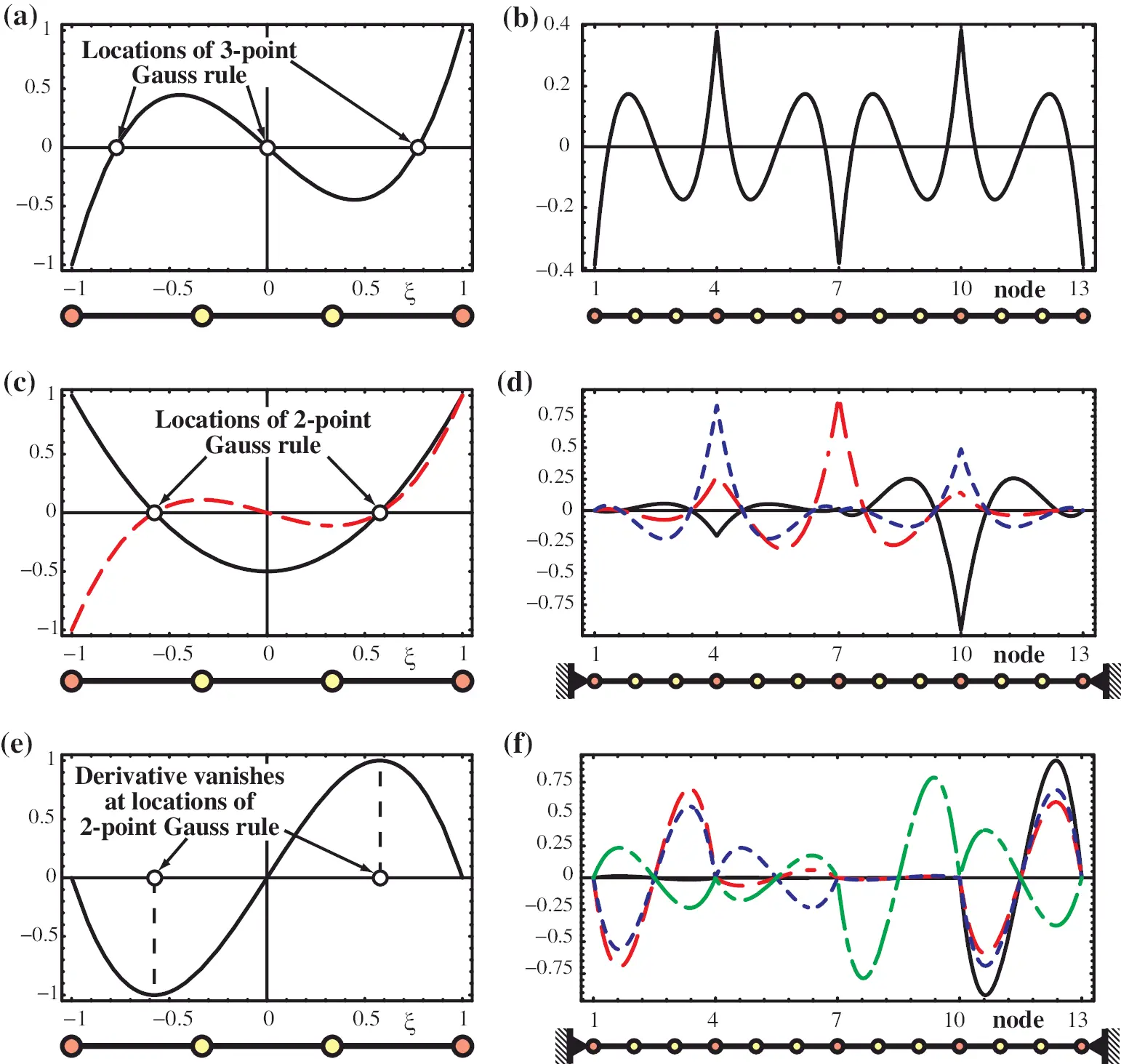Figure 3: Spurious modes in RI mass and stiffness matrices and their interelement propagation features: (a) Normalized null eigenvector of MCe(γ,3) over individual element; (b) Computed null eigenvector over a 4-element mass discretization of free-free bar modeled with MCe(γ,3); (c) Normalized null eigenvectors (symmetric and antisymmetric) of MCe(γ,2) over individual element; (d) Computed null eigenvectors over a 4-element mass discretization of fixed-fixed bar modeled with MCe(γ,2); (e) Normalized spurious null eigenvector of Ke(γ, 2) over individual element; (f) Computed null eigenvector over a 4-element mass discretization of fixed-fixed bar modeled with Ke(γ, 2). Eigenvectors displayed in (b), (d), (f) computed using Mathematica Eigensystem function; those in (d), (f) are not orthonormalized

The alleged benefits of RI in reducing DTI pollution may be negated by the presence of spurious modes. These can be benign or harmful. Three cases are considered below.

The 3-point integrated CMM of an individual element, denoted by MCe(γ,3), has an antisymmetric cubic spurious mode (the null eigenvector of MCe). This mode, denoted by uA(ξ), vanishes at the three Gauss points ξ = 0 and ξ±3/5. (Subscript A stands for antisymmetric). Normalizing so that uA = ±1 at ξ = ±1 we have

uA(ξ)=12ξ(35ξ2)., (14)

This is independent of γ. The mode is pictured in Fig. 3a. If this mass matrix is used to model a free-free bar, the mode propagates over elements in the repeating pattern illustrated in Fig. 3b. If the motion of any corner node (or of a side node if γ3/5) is set to zero, the mode disappears. Consequently it can be characterized as benign, since it stays unique no matter how many elements are assembled, and can be effectively suppressed by just one homogeneous boundary condition.

The 2-point RI mass matrix MCe(γ,2) of an individual element is rank deficient by two. The spurious modes are a linear combination of quadratic and cubic functions that vanish at the two Gauss points ξ=±1/3. It is convenient to select the following normalized null eigenvectors as basis of the null eigenspace:

uS(ξ)=12(13ξ2),uA(ξ)=12ξ(13ξ2), (15)

in which subscripts S and A stand for symmetric and antisymmetric, respectively. These are pictured in Fig. 3c. Their shapes are independent of γ. To remove the null eigenspace, both ends must be fixed. For multiple elements the modes propagate as illustrated in Fig. 3d. This plot shows three null eigenvectors for a fixed-fixed bar discretized with 4 elements; note that they vanish at the 8 Gauss points. If this problem is discretized with Ne elements, the assembled M has Ne − 1 zero eigenvalues. Thus this particular CMM may be characterized as dangerous for any γ, since spurious modes are hard to control with usual boundary conditions.

The 2-point RI stiffness matrix Ke(γ, 2) has rank deficiency of 2. The null eigenspace is spanned by

uR(ξ)=1,uA(ξ)=3(9+43)2(4+33)ξ(1ξ2)=2.59808ξ(1ξ2). (16)

Here uR(ξ) is the rigid body translational mode, which is physical. On the other hand the antisymmetric mode uA(ξ) is spurious: its derivative is zero at the Gauss points ±1/3 so associated strains vanish there. The spurious mode of (16) has been normalized so that its value is ±1 at those points, as shown in Fig. 3e. Its shape is independent of γ. Since uS(ξ) vanishes at the end nodes, it cannot be controlled by homogeneous boundary conditions there: it will “sprout” over each element of a mesh assembly. This is illustrated in Fig. 3f for a fixed-fixed bar discretized with 4 elements: the assembled stiffness has three zero eigenvalues with the eigenvectors shown. If this problem is discretized with Ne elements, the assembled K has Ne − 1 zero eigenvalues. It follows that this particular stiffness may be characterized as dangerous for any γ.

The preceding analysis applies to the mass and stiffness matrix in isolation. When combined for dynamic analysis, more complex spurious mode effects may occur.

4.5 A New Result: Gamma Invariance

The fully integrated CMM and its RI cousins display a remarkable property: the computed natural frequencies do not depend on SN location. More precisely, they are independent of γ if 0 < γ < 1. This property will be generically called γ-invariance. It can be illustrated through the frequency characteristic function (FCF) of an individual element:

Fe(γ,pM,pK,ω)=det[Ke(γ,pK)ω2MCe(γ,pM)], (17)

in which ω denotes circular frequency. Expanded FCF expressions are given in Table 3 for pM = 4, 3, 2 and pK = 3, 2. To reduce clutter E, A, ρ and l are set to 1. It can be observed that the numerators are polynomials in ω2 that do not depend on γ, while their denominators do not vanish if γ ∈ (0, 1). The natural frequencies ωi, which are roots of F = 0, are tabulated in the last column of that Table. (Note that if the reduced stiffness with pK = 2 is used, one extra root becomes zero, whereas if a reduced mass matrix is used, some roots move to because the ω-polynomial order drops). As regards vibration modes, which are the eigenvectors ϕi of the frequency eigensystem Keϕi=ωi2MCeϕi, their expressions as functions of ξ over the element are also independent of γ if their values at the SN are adjusted accordingly.

The property is somewhat surprising since the eigenvalues of MCe and Ke in isolation do depend on γ. Symbolic computations indicate that γ-invariance remains valid for arbitrary assemblies of Bar4 elements that use CMM. But it does not hold for other mass matrices such as DLMM.

Conclusion: If a CMM is used, whether FI or RI, the choice of γ makes no difference in frequency accuracy. Is there an optimal value in another sense? From a conditioning standpoint one would like to maximize the FCF denominators. As shown in Table 3, these have the form C γ2 (1 − γ2)4, in which C is a numerical coefficient independent of γ. The maximizing value in the range (0, 1) is γ=1/5, which is an interior Lobatto point of the 4-point Lobatto integration rule . This value recurs in several optimization scenarios studied later.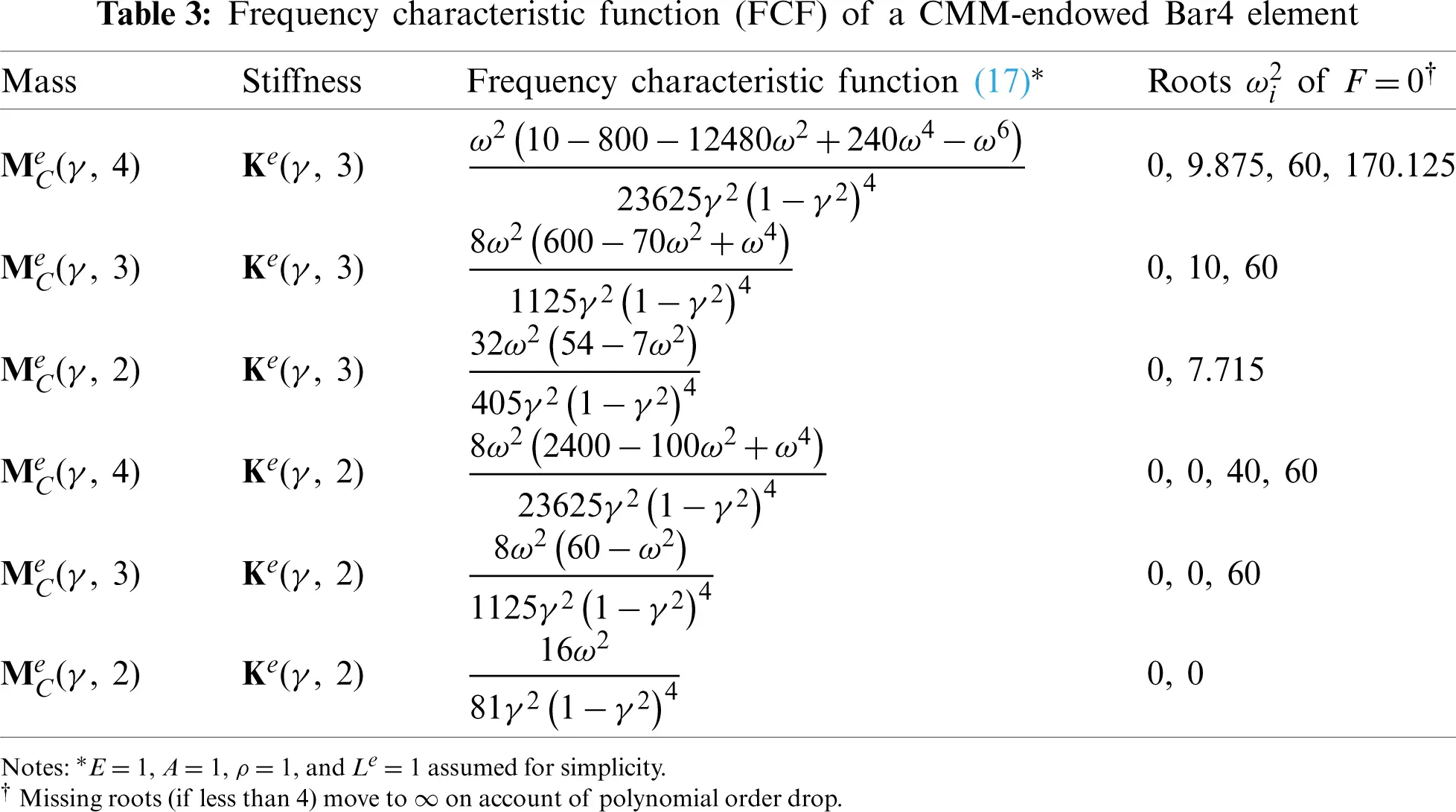4.6 Noteworthy Instances

Some mass-stiffness instances worth noting are collected here. If the interior nodes are placed at the thirdpoints, γ = 1/3 and the FI mass matrices are

MCe(13,4)=ρA1680,MLe(13,4)=ρA8, (18)

whereas the FI stiffness matrix becomes

Ke(13,3)=EA120. (19)

For this particular configuration, the DLMM displays the well known three-eighths integration formula, which is also the 4-point Newton-Cotes quadrature rule .

If the interior nodes are placed at the Lobatto points, γ=1/5 and the FI mass matrices become

MCe(15,4)=ρA84,MLe(15,4)=ρA12, (20)

whereas the FI stiffness matrix becomes

Ke(15,3)=EA12[5225(5+35)5(5+35)2525(5+35)5(5+35)5(5+35)5(5+35)100505(5+35)5(5+35)50100]. (21)

The diagonal entries and node locations of the DLMM in (20) correspond to the abcissas and weights of the 4-point Lobatto integration rule . By analogy, the foregoing matrices will be denoted as the Lobatto DLMM and Lobatto stiffness matrix, respectively.

For γ=1/10, which (as shown later) gives a near-optimal DLMM, the FI mass matrices are

MCe(110,4)=ρA3402[26239χ13χ1439262χ14χ13χ13χ141360240χ14χ132401360],MLe(15,4)=ρA20, (22)

in which χ13=70+4610 and χ14=704610.

5  Plane Wave Propagation over Bar4 Lattice

We consider the propagation of plane waves over an infinite lattice of identical Bar4 elements obtained by space discretization of a continuum bar model. Fig. 4 is a schematics of the continuum-to-lattice semi-discretization process. The formulation concludes with the extraction of a two-element patch about a typical end node n. The necessary terminology is collected in Table 4.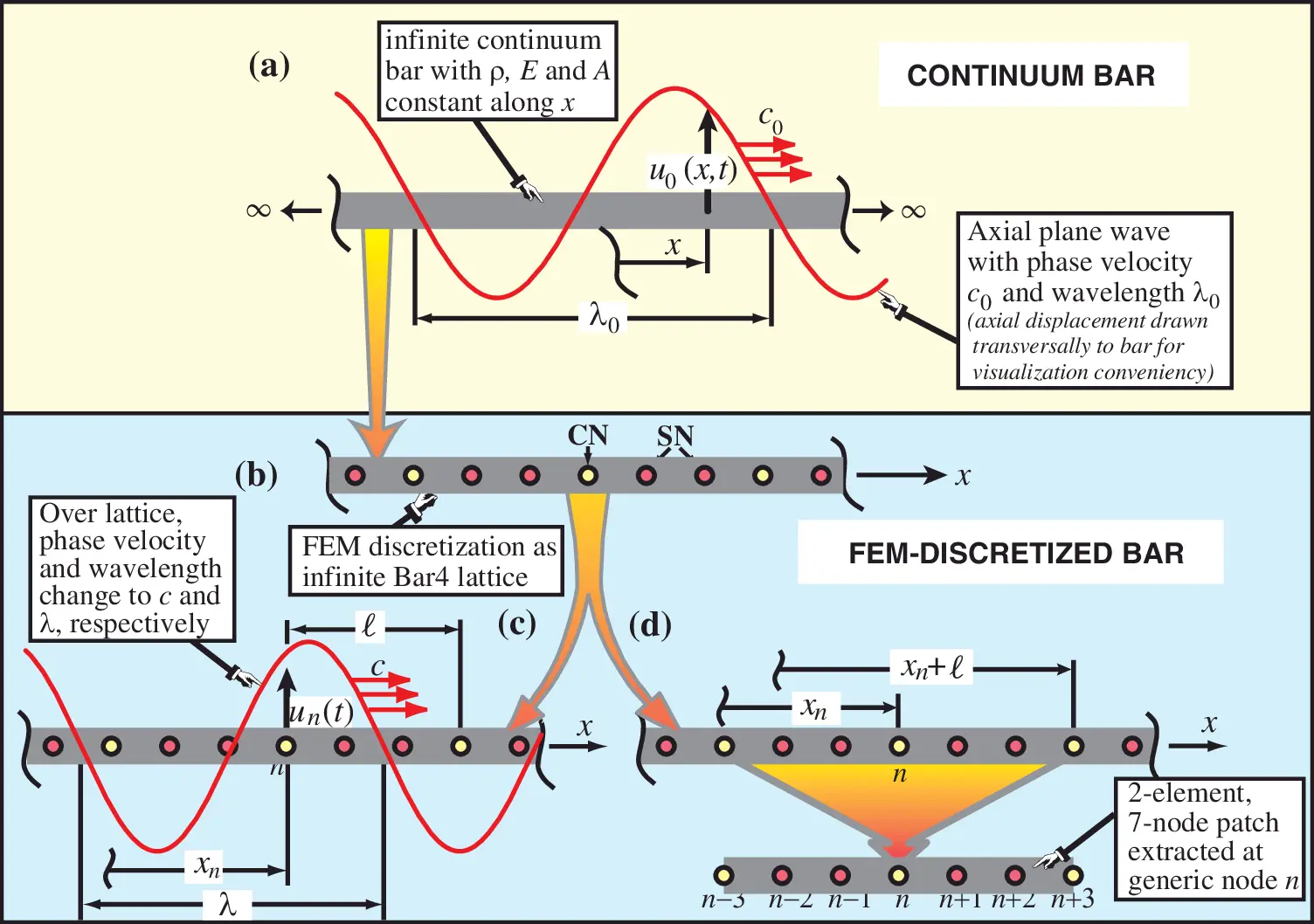Figure 4: Schematics of plane wave fourier analysis: (a) Continuum bar model; (b) FEM discretization as Bar4 lattice; (c) Propagation of plane wave over lattice; (d) Extraction of two-element, seven-node patch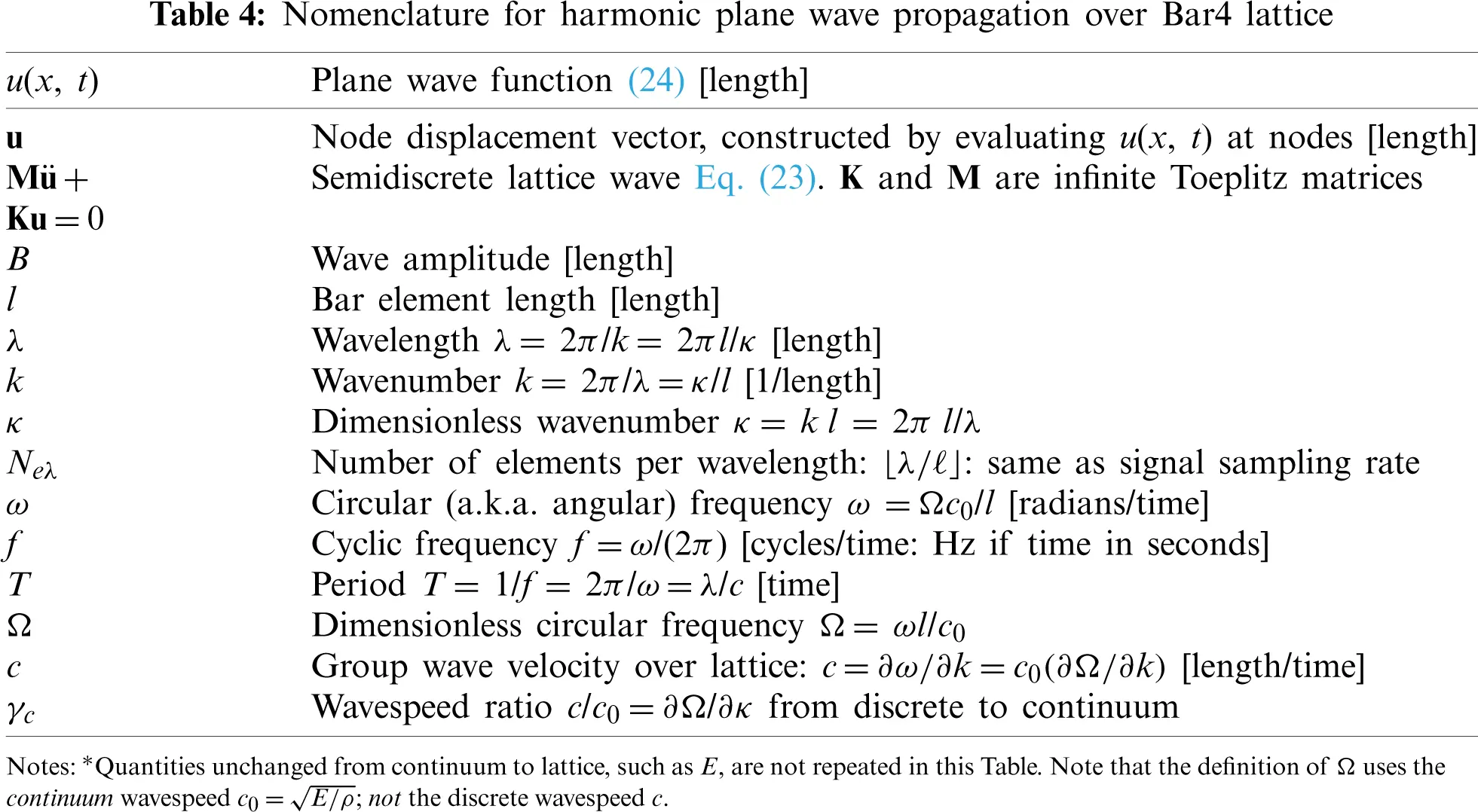5.1 Plane Wave Equations

Plane wave propagation over a regular spring-mass lattice is governed by the semidiscrete, linear equation of motion (EOM):

Mu¨+Ku=0. (23)

Here u is the vector of axial nodal displacements while M and K are infinite, tridiagonal, Toeplitz mass and stiffness matrices. This EOM can be solved by Fourier methods. Fig. 4b displays two characteristic lengths: λ and l. The element length-to-wavelength ratio is called . The floor function of its inverse: Neλ=λ/ is the number of elements per wavelengths. Those ratios characterize the fineness of the discretization, as pictured in Fig. 4b.

Within constraints noted later the lattice can propagate real, travelling, harmonic plane waves of wavelength λ and group velocity c, as depicted in Figs. 4b, 4c. The wavenumber is k = 2π/λ and the circular frequency ω = 2π/T = 2π c/λ = k c. The range of physical wavelengths that the lattice may transport is illustrated in Fig. 5.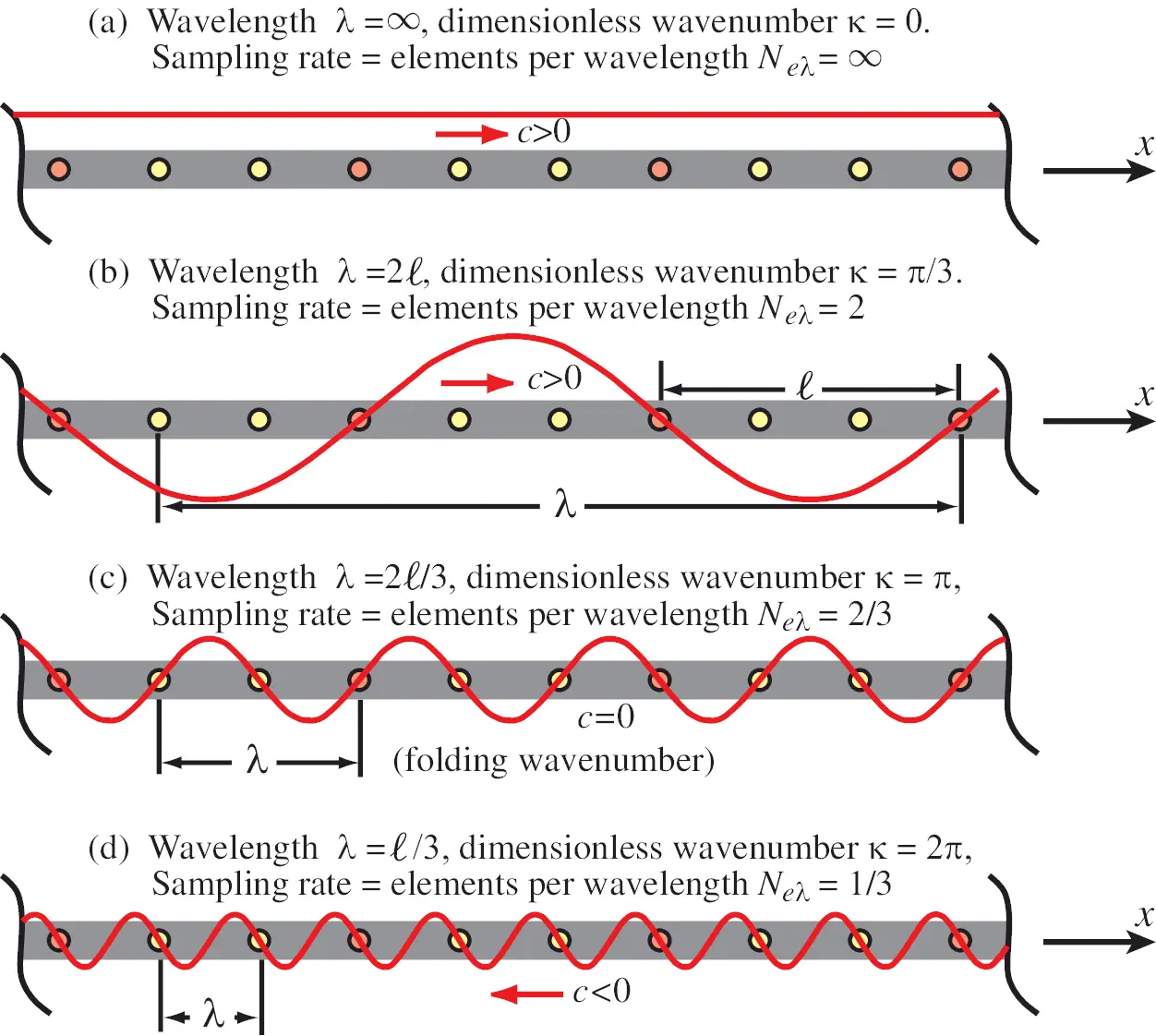Figure 5: Plane waves of various wavelengths that a Bar4 lattice may transport. Yellow and red circles mark corner and side nodes, respectively

To study plane wave solutions of (23) it is sufficient to extract a repeating two-element patch, a process schematized in Fig. 4d. The seven patch nodes are renumbered as shown in Fig. 6, and the x axis origin relocated to the interface node n. The natural patch coordinate ξ^=x/ is defined as shown in the figure. A harmonic plane wave of amplitude B is described by the function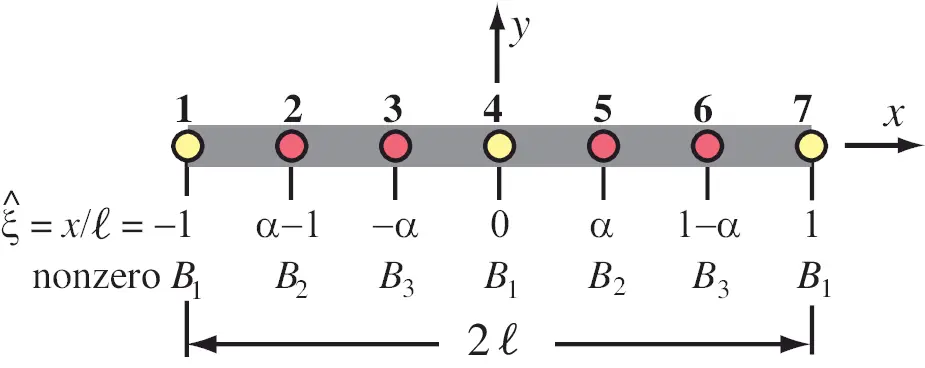Figure 6: Two-element Bar4 patch that shows: renumbered nodes, x-axis origin relocated to interface node 4, natural patch coordinate ξ^=x/, and the amplitude coefficients Bi of the plane wave expansion (29) that are nonzero at the indicated nodes

uB(x,t)=Bexp[j(kxωt)]=uB(ξ^,τ)=Bexp[j(κξ^Ωτ)],j=1. (24)

In the second form the dimensionless wavenumber κ, circular frequency ω, and time τ are defined as κ = k l = 2πl/λ, ω = ω l/c0, and τ = tc0/l, respectively. Here c0=E/ρ is the elastic continuum bar group wave velocity, which for that model is the same as the phase velocity. (In physical acoustics c0 is the sound speed of the material.) In both forms B has dimension of displacement.

Using the stiffness and mass matrix notation of (11)(13) the patch equations can be written

MPu¨P+KPu=0, (25)

in which

uP=[u1 u2 u3 u4 u5 u6 u7]T,

MP=ρA[χ11χ13χ14χ12000χ13χ33χ34χ23000χ14χ34χ44χ24000χ12χ13χ24χ11+χ22χ13χ14χ12000χ13χ33χ34χ23000χ14χ34χ44χ24000χ12χ23χ24χ22], (26)

KP=EA[ψ11ψ13ψ14ψ12000ψ13ψ33ψ34ψ23000ψ14ψ34ψ44ψ24000ψ12ψ13ψ24ψ11+ψ22ψ13ψ24ψ12000ψ13ψ33ψ34ψ23000ψ14ψ34ψ44ψ24000ψ12ψ23ψ24ψ22].

Here the χij and ψij are the dimensionless coefficients introduced in (11) and (13). (The extra subscript C is suppressed since this form applies to all admissible mass and stiffness matrices.) From the patch EOM (25) take the middle 3 equations (Rows 3, 4 and 5 of MP and KP), which repeat in the infinite lattice:

M^Pu¨P+K^PuP=0. (27)

Here M^P and K^P are 3 × 7 matrices.

5.2 Fourier Analysis

The axial wave motion is represented using three basis functions: uB1(ξ, τ), uB2(ξ, τ) and uB3(ξ, τ) with the same structure as the last term in (24)

uB(ξ^,τ)=i=13uBi(ξ^,τ),in whichuBi(ξ^,τ)=Biexp[j(κξ^Ωτ)],i=1,2,3. (28)

Coefficients Bi are linked to B by

Bi(ξ^)=defBgi(ξ^),i=1,2,3, (29)

in which gi(ξ^) are functions of patch position, chosen so they are linearly independent and none is identically zero. For future use the Bi are grouped into the column 3-vector B = B1 B2 B3T, and the gi into the 3 × 3 diagonal matrix G = diag[g1, g2, g3], whence B = GB.

For convenient implementation we pick the gi as sketched in Fig. 6, namely B1 vanishes at all nodes but 1, 4 and 7, B2 vanishes at all nodes but 2 and 5, and B3 vanishes at all nodes but 3 and 6. There is an infinite number of such gi functions. However, their actual form is irrelevant because the eigenvalues of the characteristic system (36) derived below do not depend upon the gi(ξ^). Accordingly, the nodal patch displacement vector is set as follows

uP=[B1exp[j(κΩτ)]B2exp[j(κ(1γ)Ωτ)]B3exp[j(κγΩτ)]B1exp[Ωτ)]B2exp[j(κγΩτ)]B3exp[j(κ(1γ)Ωτ)]B1exp[j(κΩτ)]]. (30)

Substituting (30) into (27) yields the wave propagation condition

[C11C12C13C11C12C13C11C12C13][g1000g2000g3][C11C12C13C11C12C13C11C12C13]B=[B1B2B3]=CB==0. (31)

If C B = for B ≠ 0, C must be singular. This furnishes the characteristic equation det(C) = 0, from which factors depending on τ only may be dropped. The dimensionless frequency Ω appears only in even powers; more precisely 2, 4 and 6. It is convenient to define Ω˜=Ω2 as new variable. Then det(C) = 0 is a (complicated) cubic equation in Ω˜ that provides three solutions denoted as Ω˜1=Ω12, Ω˜2=Ω22, and Ω˜3=Ω32. One of those vanishes at κ = 0 and is renamed a for acoustic branch. The remaining two roots correspond to optical branches and are relabeled Ωo12 and Ωo22, which are ordered so Ωo12Ωo22 at κ = 0 (these are the so called cutoff frequencies). When expressed as functions of κ:

Ωa2=Ωa2(κ),Ωo12=Ωo12(κ),Ωo22=Ωo22(κ), (32)

these represent the equations of the acoustic and optical branches. The combined plot of these branches as Ω(κ) curves is called the dimensionless dispersion diagram or DDD. This is done for the Brillouin zone κ ∈ [0, 2π].

6  Low Frequency Performance

The dimensionless series expansions of the acoustic branch about κ = 0 is written

Ωa2=κ2+A44!κ4+A66!κ6+A86!κ8+, (33)

whereas those of the optical branches are

Ωo12=Ωo102+B22!κ2+B44!κ4,Ωo22=Ωo202+C22!κ2+C44!κ4+ (34)

The performance of a mass-stiffness (MS) pair in structural dynamics is controlled by the accuracy of the acoustic branch for low frequencies, i.e., small wavenumbers. Since the acoustic branch for the continuum is Ωa = κ, or Ωa2=κ2, the accuracy is controlled by the first nonzero term after κ2 in the 2a series (33). The will be called the principal error term, or PET, in the sequel. For example if A4 = 0 and A6 = −24, the PET is A6 κ6/6! = −κ6/30.

6.1 Performance of CMM and DLMM

Table 5 shows the coefficients of (33) for fully integrated (pM = 4) CMM mass matrices, as well as two RI instances obtained with pM = 2 and 3 Gauss points. The stiffness matrix is either the exactly integrated one with pK = 3 or the RI instance with pK = 2. As can be observed the expansion does not depend on γ, confirming the γ-invariance analysis of Section 4.5. Thus the accuracy order displayed in the last column cannot be improved by moving nodes.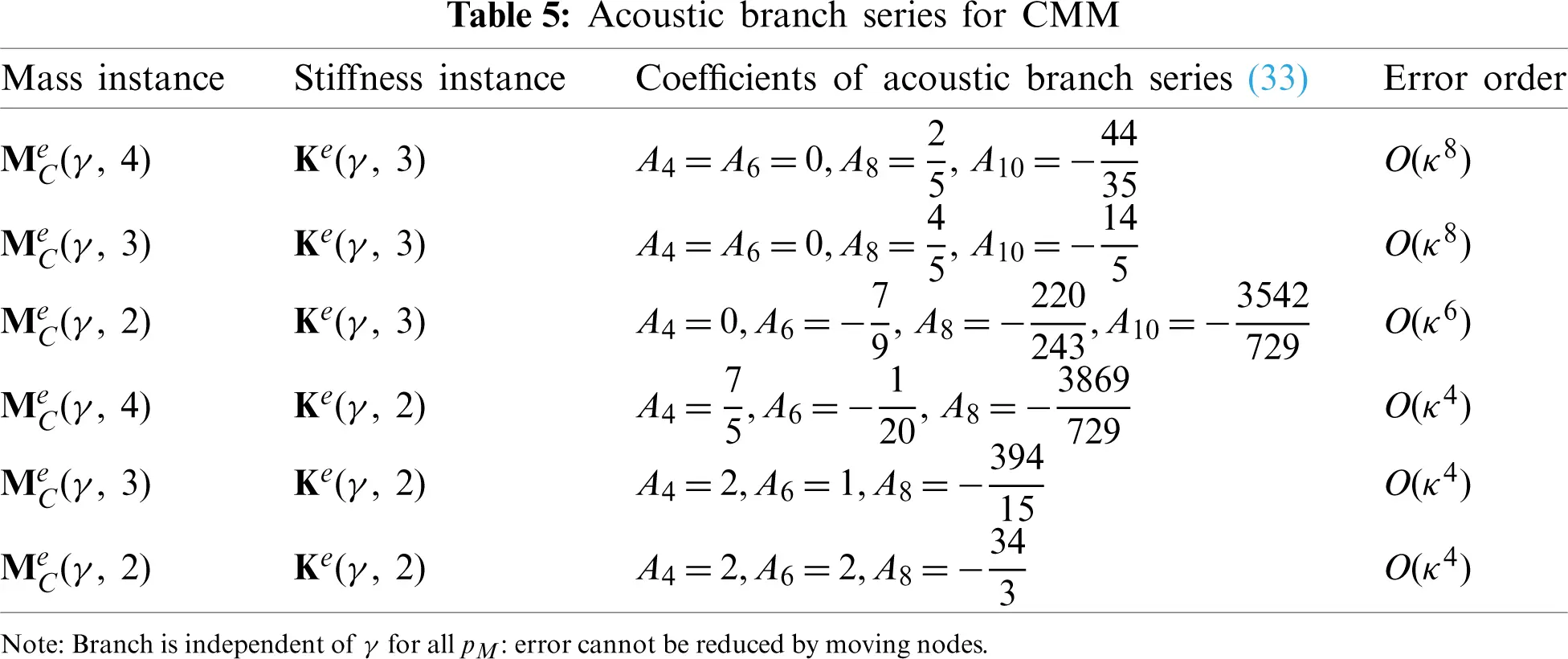Table 6 shows the coefficients of (33) for DLMM mass matrices. These matrices are identical for pM = 2, 3, 4, as shown in Table 1. Consequently only two rows are needed in Table 6 for ML(γ, pM) paired with the FI and RI stiffness matrices. The series coefficients now depend on γ—more precisely its even powers. The first row show that the pairing of Me(γ, pM) with Ke(γ, 3) has generally accuracy order O(κ6). This may be elevated to O(κ8) by solving −2A6 = 1 − 15γ2 + 50γ4 = 0. This yields two solutions: γ2B1 = 1/5 and γ2B2 = 1/10, in which subscript B stands for “best.” Taking the positive square roots delivers the DLMM-optimal node locations

γB1=15=0.4472135954999579,γB2=110=0.31622776601683794. (35)

The corresponding PETs are −(2/15) κ8/8! and −109/240 κ8/8!, respectively. The first coefficient is about 3 times smaller (actually 109/32 ≈ 3.406 smaller). Thus the internal node location γB1=1/5, which corresponds to the 4-point Lobatto rule, is optimal for DLMM together with Ke(γ, 3). Note that if γ = 1/3, the PET is +(4/81) κ6/6!, which for κ = 1 is about 151 times bigger than optimal. As regards the RI stiffness matrix Ke(γ, 2), the error order O(κ4) may not be raised by solving A4 = 4γ2/(1 − γ2) = 0 because γ cannot vanish.Dimensionless dispersion diagrams for various instances of CMM and DLMM are shown in Figs. 7 and 8, respectively.6.2 Performance of CMM-DLMM Combinations

The truncation error −(2/15) κ8/8! for the optimal DLMM with γ=1/5 and pM = 2, 3, 4 is smaller than that of the exactly integrated CMM, which is (2/5) κ8/8!, and of opposite sign. Consequently a linear combination of those matrices, henceforth denoted by LCMM, looks promising as far as elevating the error order to O(κ10). The combination weights are μ and 1\,\,−\,\,μ, where μ is another template parameter. This follows the notation of .

Table 7 lists coefficients (computed by Mathematica) of the acoustic branch series (33) for the two-parameter mass matrix combinations

MLCe(γ,pM,μ)=(1μ)MCe(γ,pM)+μMLe(γ,pM),pM=2,3,4,μ[0,1], (36)

paired with Ke(γ,pK) for pK = 3,2. The catalogued coefficients extend up to A10 for pK = 3, and to A6 for pK = 2, in accordance to the expected principal error terms (PET).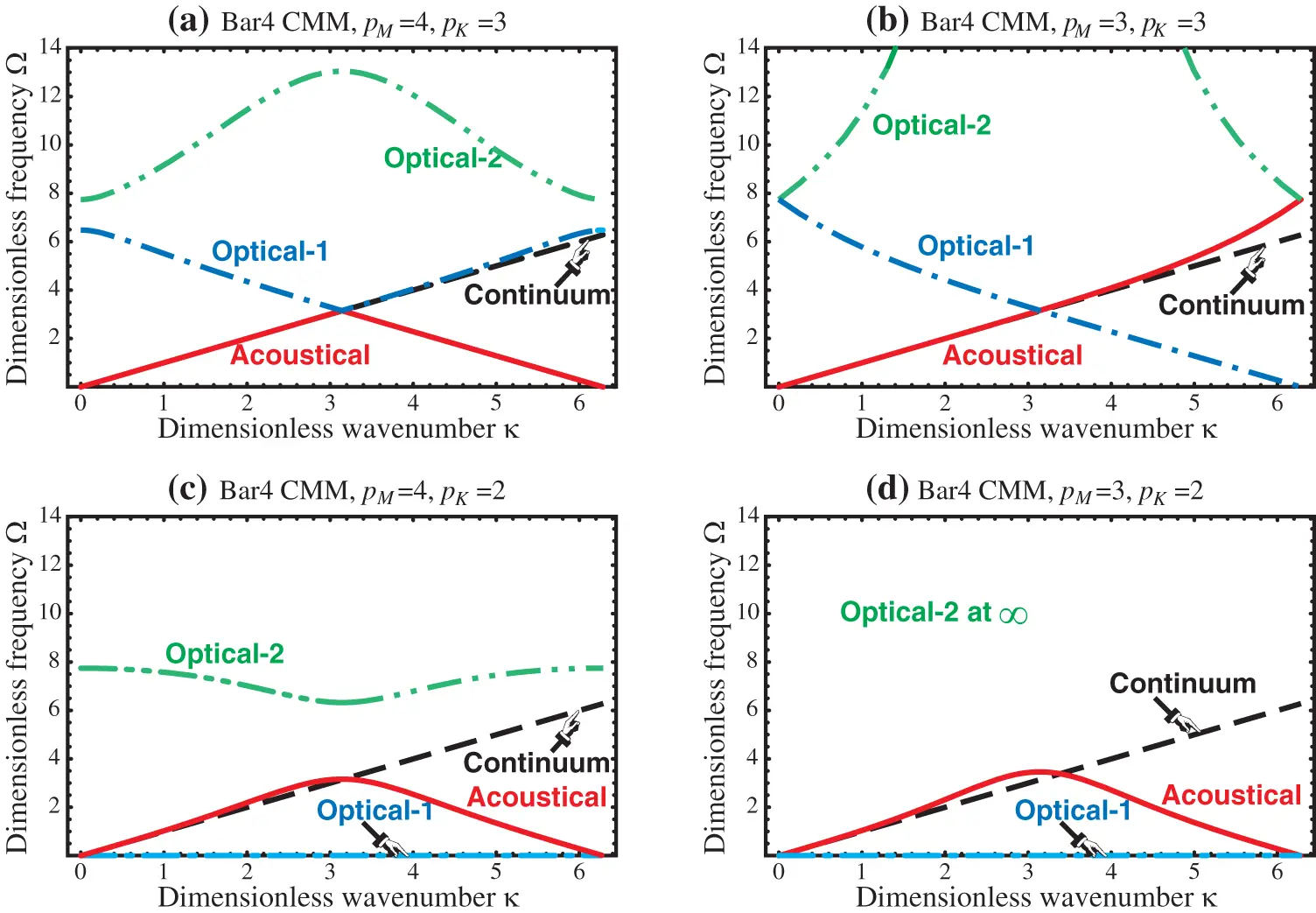Figure 7: Dimensionless dispersion diagrams (DDD) of several Bar4 combinations of full and reduced-integration consistent-mass and stiffness matrices: (a) MeC(γ, 4) and Ke(γ, 3); (b) MeC(γ, 3) and Ke(γ, 3); (c) MeC(γ, 4) and Ke(γ, 2); (d) MeC(γ, 3) and Ke(γ, 2). The value of γ ∈ (0, 1) is irrelevant

If the stiffness matrix is fully integrated (pK = 3), a PET of O(κ10) can be obtained by setting A6 = A8 = 0 and solving for γ2 and μ. This produces a system of two equations, cubic in γ2 and linear in μ. Of the three solutions, one gives negative γ2, and another one yields an indefinite LCMM. The remaining one is

pM=4:γ2=1/5,μ=3/4,

pM=3:γ2=1/5,μ=6/7, (37)

pM=2:γ2=4/35,μ=14/23.

On taking the positive square root, we get the optimal locations γ=1/5 for pM = 3, 4 and γ=2/35 for pM = 2. The latter: γ≈0.338062 is very close to 1/3. All three MS pairs have exactly the same PET: −(2/15) κ10/10!, as may be verified. The optimal LCMM associated with the 3- and 4-point Gauss rules actually coalesce: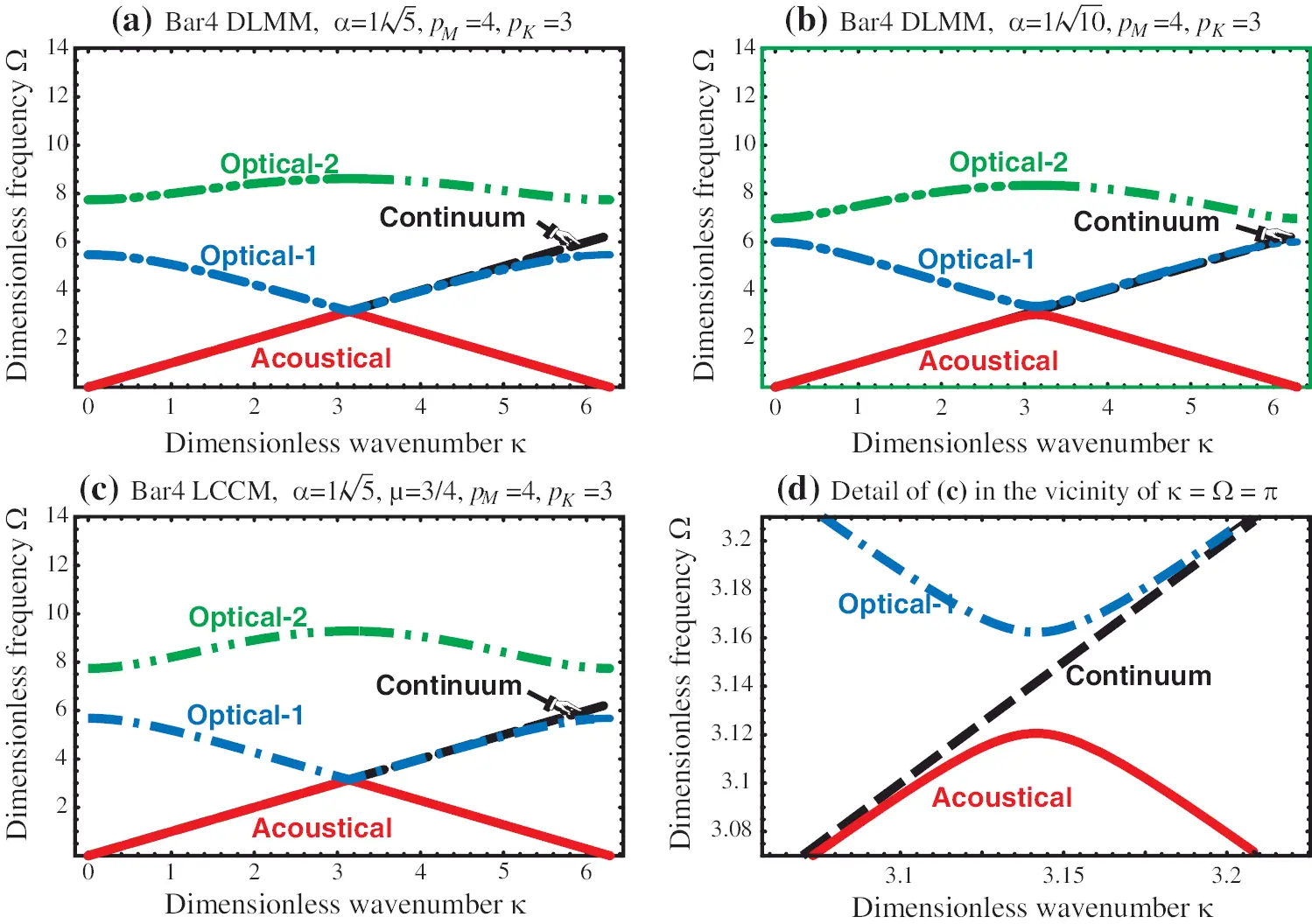Figure 8: Dimensionless dispersion diagrams (DDD) of optimal Bar4 DLMM and LCMM instances paired with the fully integrated stiffness matrix. (a) MeL(1/5, 4) and Ke(γ, 3); (b) MeL(1/10, 4) and Ke(γ, 3); (c) MeLC(1/10, 4, 3/4) and Ke(γ, 3); (d) Detail of (c) Near k = Ω = p (Nyquist frequency and wavelength)

MLCe(15,4,34)=MLCe(15,3,67)=ρAl336[ 2715512755551355555135 ]=ρAl[ 0.080357140.002976190.006654960.006654960.002976190.080357140.006654960.006654960.006654960.006654960.401785710.014880950.006654960.006654960.014880950.40178571 ] (38)

The optimal LCMM associated with the 2-point rule is different:

MLCe(15,4,34)=MLCe(15,3,67)=ρAl336[ 271551275555135555513MLCe(235,2,1423)=ρAl265236[ 220801058ϕ1ϕ2105822080ϕ2ϕ1ϕ1ϕ211833528175ϕ2ϕ128175118335 ]80pt=ρAl[ 0.083246620.003988900.036165690.000254700.003988900.083246610.000254700.036165690.036165690.000254700.446149840.106226150.000254700.036165690.106226150.44614984 ]5 ]=ρAl[ 0.080357140.002976190.006654960.006654960.002976190.080357140.006654960.006654960.006654960.006654960.401785710.014880950.006654960.006654960.014880950.40178571 ] (39)

in which ϕ1=805(6+35) and ϕ2=805(6+35). Both (38) and (39) have positive eigenvalues; whence they are admissible.

As regards the RI stiffness with pK = 2, order elevation to O(κ8) requires A4 = A6, a system that lacks solutions. Thus only O(κ6) may be attempted by setting A4 = 0, which leads to a quadratic equation in γ2. The solution γ=±μ1/(5μ) gives imaginary γ for μ ∈ (0, 1).

7  Reducing Numerical Pollution

In structural dynamics, optical branches have no physical meaning. They are a byproduct of the superlinear spatial discretization. Their presence is harmless in vibration analysis or in transient response computations carried out via mode superposition, as they are automatically filtered by the eigensolver. In direct time integration (DTI), however, thay can be responsible for “numerical pollution” that can render the computed solution worthless. In fact, this is the main reason for preferring low-order elements in that case, since those do not carry along that harmful extra baggage.

If one is determined to use elements such as Bar4 in DTI, pollution may be reduced by moving or flattening optical branches so that acoustoptical gaps are widened, because noise that falls into those gaps does not propagate. Several techniques for doing that are discussed in detail in  for the 3-node bar. Here we simply consider the effect of reduced integration on the position of optical branches. For a quick assessment we look at the so-called cutoff frequencies, which are the values taken by the optical branches at zero wavelength: κ = 0. Those are denoted by Ωc1=Ωo1|κ0 and Ωc2=Ωo2|κ0, ordered so that Ωc1 ≤ Ωc2.

Table 8 gives cutoff frequency expressions for parametrized LCMM-stiffness pairs, including fully integrated (FI) as well as several reduced integration (RI) versions. Table 9 lists numerical values for selected mass-stiffness pair instances, again showing the effect of the integration rule.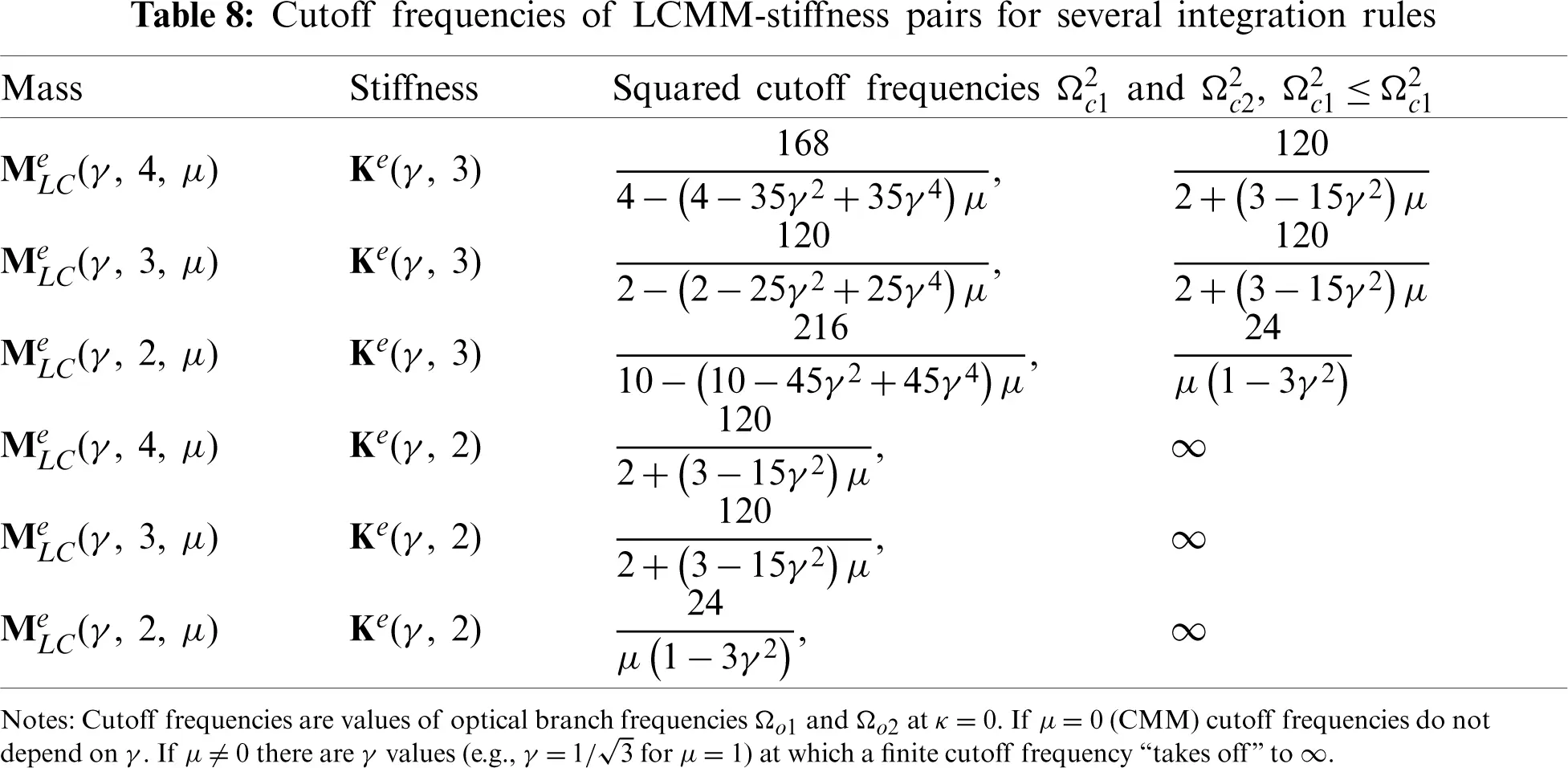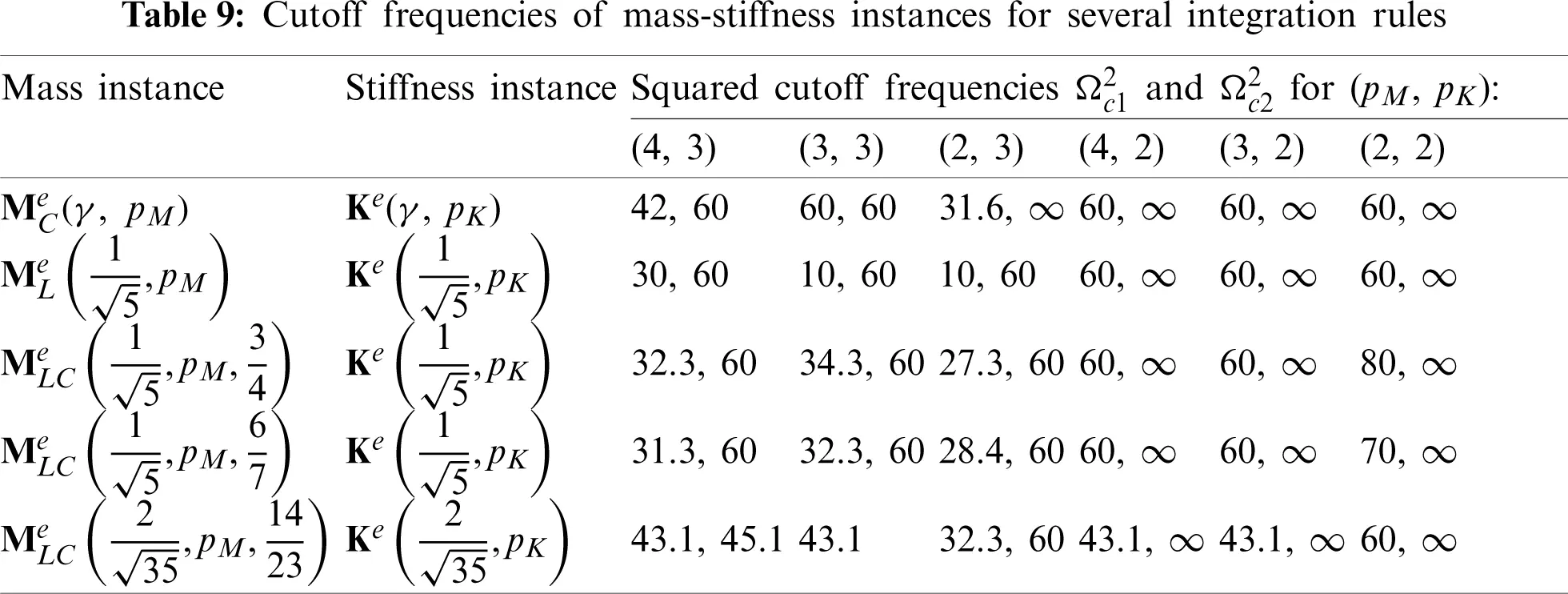An effective way to cut pollution is to move an optical branch to infinity. As Table 8 shows, this can be done by using a RI stiffness paired with any mass matrix. To get rid of the other branch one may set γ and μ so that its cutoff frequency denominator vanishes. For example μ = 1 and γ=1/3 (which places the SN at the 2-point Gauss locations) gets rid of both optical branches. Inevitably this reduces low frequency accuracy, which is only O(κ4) as shown in Section 6.2. In that case (μ = 1 and γ=1/3) the acoustic branch PET is down to κ4/12. This is worse that the best-fit instance of the much simpler 2-node bar element, which does not possess an optical branch.

To maintain low-frequency accuracy while alleviating pollution it would be necessary to consider more general mass templates, as done in  for the 3-node bar element. RI is insufficiently powerful to accomplish that goal. Such study has not been carried out.

8  Bar4 Test: Vibrations of a Fixed-Free Bar Member

Natural frequency predictions using several Bar4 mass instances are compared for predicting the first 3 natural frequencies of longitudinal vibrations of the fixed-free elastic bar member pictured in Fig. 9. The member is prismatic, with constant E = 1, A = 1, and ρ = 1. The total member length is taken as L = π/2 for convenience. With those numerical properties the continuum eigenfrequencies are

ωi=(2i1)π2LEρ=2i1,i=1,2,3, (40)

The member is divided into Ne identical elements, with Ne = 1, 2, … 8. Fig. 9a illustrates the case Ne = 4. Five template instances, identified in Table 10, are tested. Both mass and stiffness are fully integrated. Numerical results obtained for the first three frequencies are collected there. The higher convergence of BLCD is obvious. For example, 2 elements give ω2 correct to 4 digits while both CMM and DLMM give only 2. CMM, SDMM and LDMM overestimate frequencies whereas TDMM and BLCD underestimates them.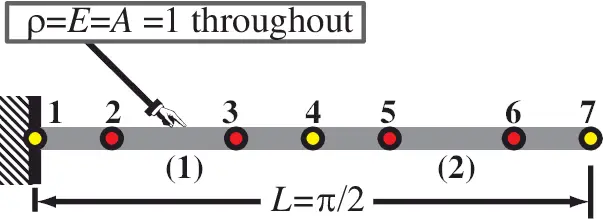Figure 9: Fixed-free homogeneous prismatic elastic bar member used in vibration test for several Bar4 mass matrix instances. The figure shows a 2-element discretization with 7 nodes. Side nodes 2, 3, 5, and 6 are drawn at the lobatto pointsThe results of Table 10 are graphically reformatted in Fig. 10, as log-log plots that display accuracy vs. element count. The horizontal axis shows number of elements Ne in log2 scale. The vertical axis displays correct digits of computed frequency, computed as

d=log10|Δωi|,inwhichΔωi=ωiFEMωi. (41)

Here Δωi is the frequency error of computed values ωiFEM with respect to the continuum frequencies (40). Note that accuracy-log plots such as those in Fig. 10 are unable to show whether the convergence is from above or below, because of the taking of absolute values in (41). That visualization deficiency should be kept in mind should frequency bounding be important. The plots clearly show at a glance that, for the same Ne, BLCD roughly doubles the number of correct digits provided by the other instances. It also illustrates that CMM and LDMM gave the same results, while SDMM is the worst performer.Figure 10: Performance of selected Bar4 template instances in predicting the first three natural frequencies ωi, i = 1, 2, 3 of the fixed-free prismatic homogeneous bar shown in Fig. 9a. This is a graphical, log-log representation of the results of Table 10. Horizontal axis shows number of elements while vertical axis displays correct digits of computed frequency. See (41) for the definition of d

9  Two-Dimensional Elements

This section extends some of the previous ideas to two-dimensional plane stress elements. A comprehensive symbolic Fourier analysis of higher order 2D elements of arbitrary geometry is presently beyond the capability of computer algebra systems. In the interest of brevity, the study is limited to a simple vibration benchmark, from which some preliminary conclusions are drawn.

9.1 The (n − 1)/n Conjecture

For bar elements with n ≤ 4 nodes, the optimal combination of the consistent and HRZ-lumped mass matrices (in the sense of best fit to the acoustic branch) has been found to be

MLC,best=1nMC+n1nML,n=2,3,4. (42)

For the linear element (n  = 2) this result was first presented in  and corroborated in . For the quadratic element (n  = 3) see [11,9]. For the present cubic element (n  = 4) see Section 6.1. Whether (42) holds for n > 4 has not been investigated. Confirmation would have limited practical value, however, since shape functions beyond cubic are rarely used in dynamics. It does suggest, however, that lumped mass matrices perform relatively better as the order rises.

More interesting is the question: Can (42) be extended to two dimensional elements? The numerical study that follows suggest that the extension is only partly correct.

9.2 Cantilever Vibration Benchmark

The benchmark 2D problem concerns the lowest fundamental frequencies of the slender 8:1 cantilever beam shown in Fig. 11a. The beam has a narrow rectangular cross section. It is fabricated of isotropic material with E = 2880, ν = 0, and ρ = 1. The dimensions shown in the Figure are: beam height H = 1, beam span L = 8, and cross section width h = 1.

Regular meshes of square elements are considered. The number Nx of elements along the span varies from 8 through 64 while the number Ny through the height varies from 1 through 8. A 16 × 2 mesh is shown in Fig. 11b. The root clamping condition is imposed by setting ux and uy to zero at all root nodes.Figure 11: Cantilever vibration benchmark: (a) Structure; (b) A 16 × 2 FEM mesh idealization (nodes not pictured)

The first six lowest natural frequencies are listed in Table 11. They comprise four flexural (bending) modes with circular frequencies ω1, ω2, ω4 and ω5, as well as two bar-like (axial, longitudinal) modes: ω3 and ω6. It should be noted that flexural frequencies given by the Bernoulli-Euler and Timoshenko beam models differ markedly from those listed there because of 1D behavioral approximations as well as neglect of root clamping details. The associated mode shapes are pictured in Fig. 12.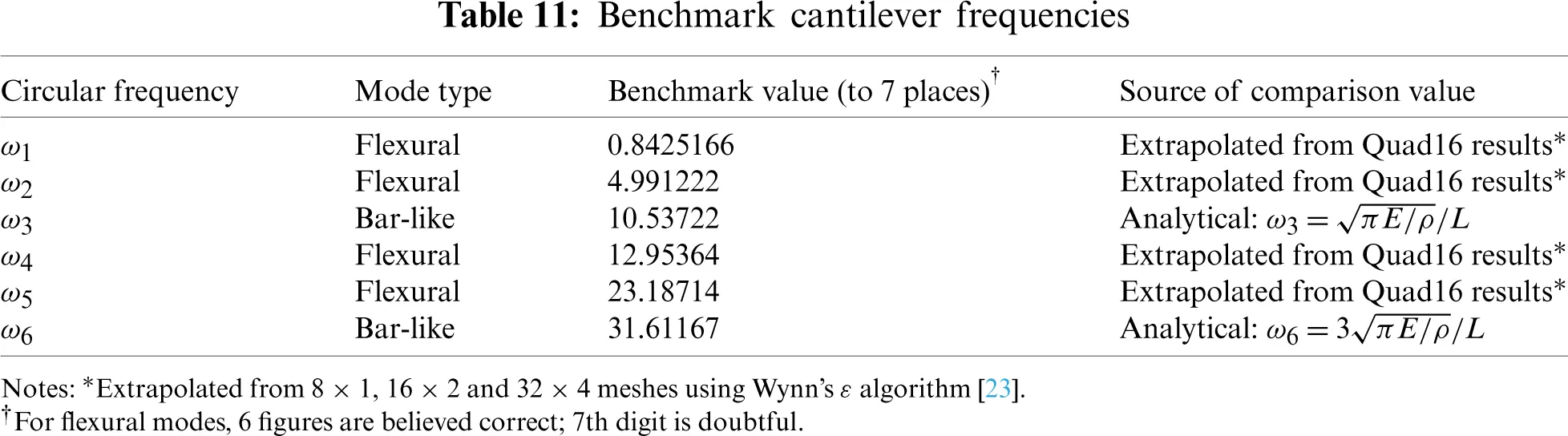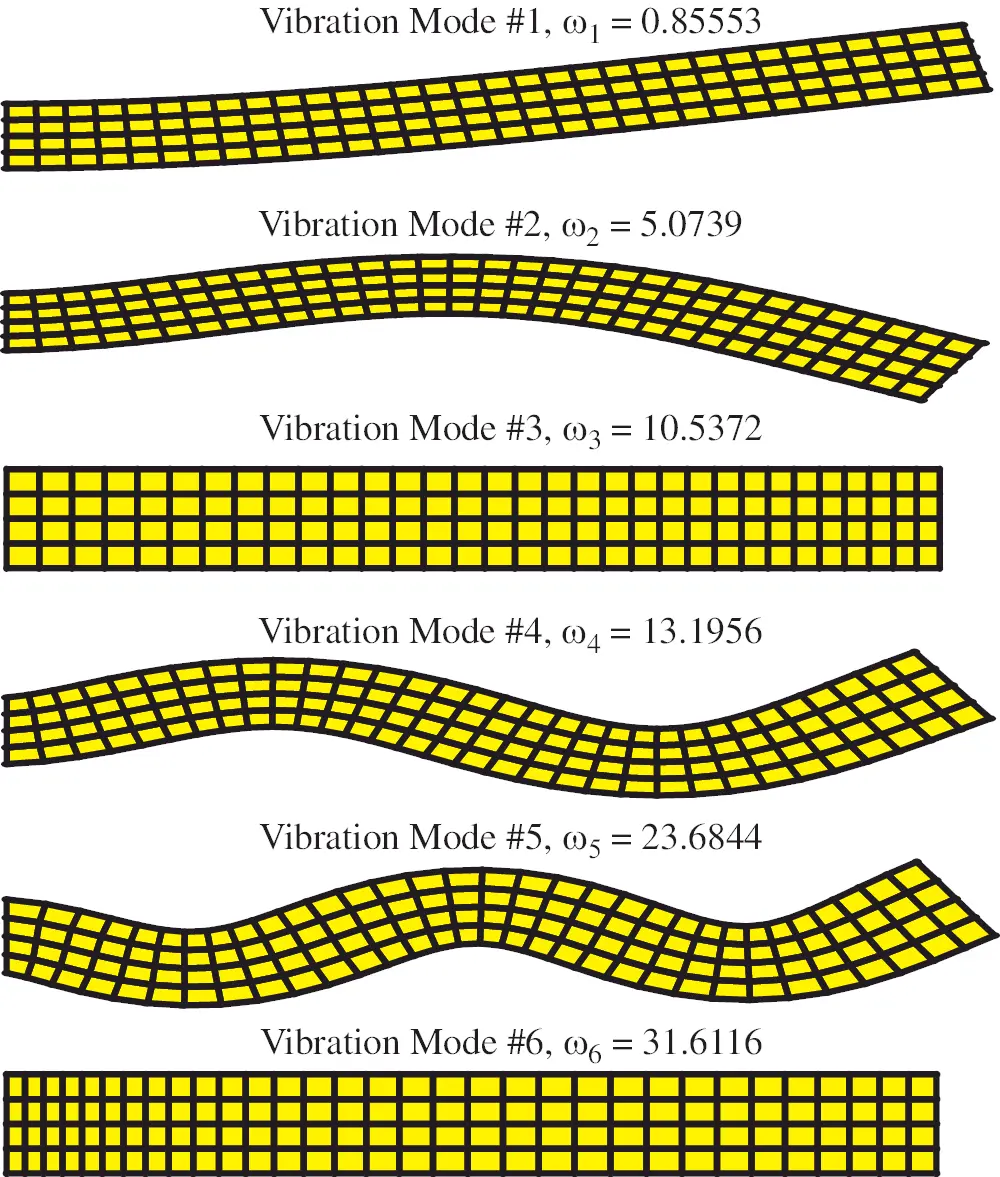Figure 12: First six vibration modes for problem of Fig. 11 obtained with a 32 × 4 Quad4 FEM discretization. Displacements grossly exaggerated for visibility

9.3 Plane Stress Finite Element Modeling

The benchmark cantilever problem was modeled with regular FEM plane stress meshes ranging from 8 × 1 through 64 × 8. Three standard Lagrangian isoparametric models were tested:

•   Quad16: bicubic quadrilateral, 16 nodes, 32 DOF, pictured in Fig. 1c.

Frequencies for the first six lowest frequency modes (those depicted in Fig. 12) were compared to the benchmark frequencies in Table 11. The relative accuracy is measured by

d=log10|ωiFEMωiωi|. (43)

in which ωiFEM and ωi are the computed and benchmark frequencies, respectively, and i ranges from 1 to 6. The value d indicates the number of significant digits obtained. As noted in Section 8 this measure cancels out the error sign. However, in the present benchmark the convergence is often (but not always) monotonic.

9.4 Plane Stress Finite Element Results

For the Quad4 models, the mass matrix is taken as the LCMM

MLCe(γ,pM,μ)=(1μ)MCe+μMLe. (44)

in which MC is computed with full integration and ML is its HRZ-lumped form. Six values of μ are tested: 0 (consistent mass), 1/4, 1/2, 2/3, 3/4, and 1 (lumped mass). The results are presented in Fig. 13. For the two bar-like modes (#3 and #6) the superior convergence of μ = 1/2 is obvious, in agreement with (42). For the four flexural modes, the convergence rate is roughly similar for all μ tested, with μ = 1 (lumped mass) consistently providing the best result and μ = 0 (consistent mass) the worst. The advantage is insignificant for the fundamental mode but becomes more visible as the frequency increases.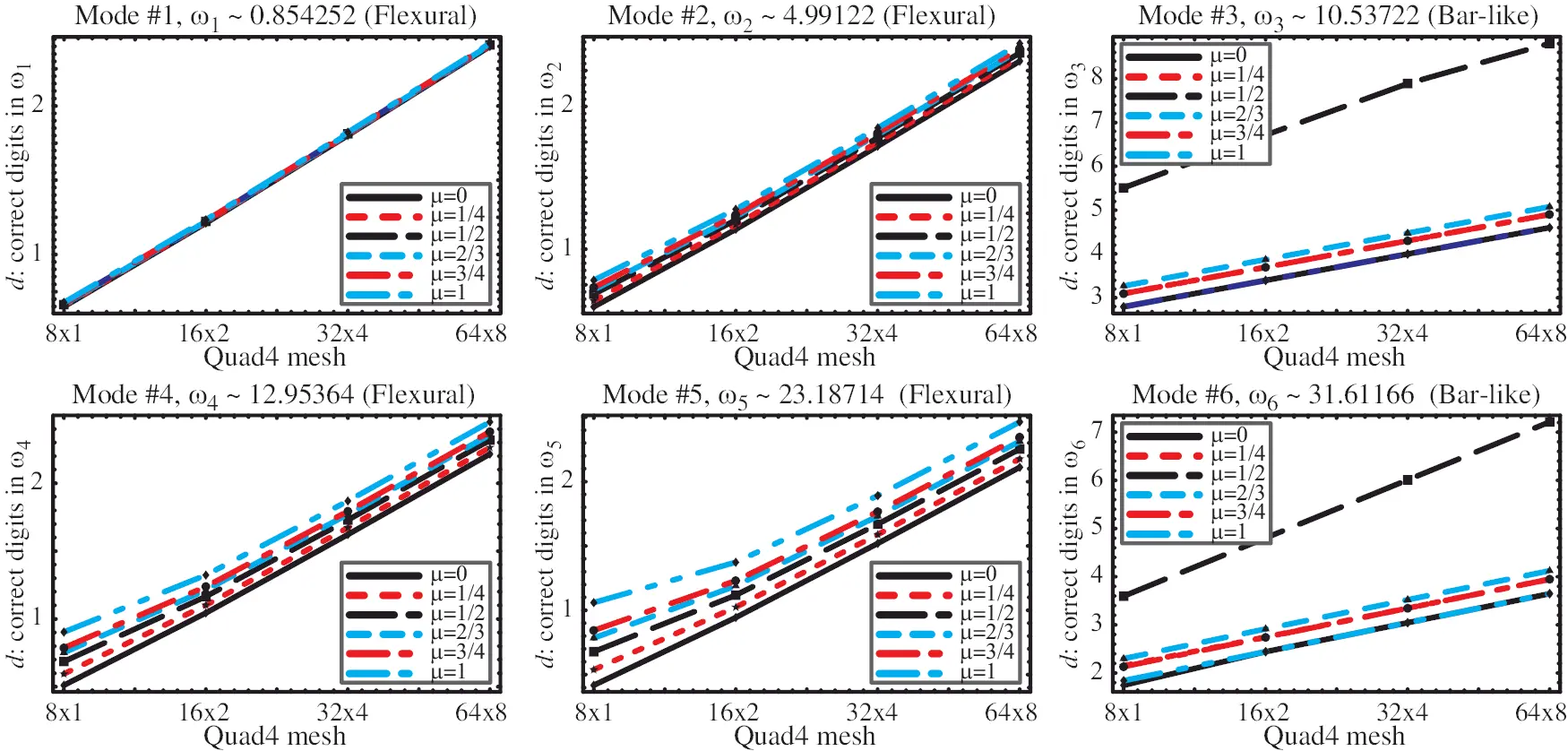Figure 13: Accuracy of computed Quad4 frequencies for four meshes (8 × 1 through 64 × 8) as affected by parameter μ of the consistent-lumped mass combination MLC = (1 − μ)MC + μML

For Quad9 the LC combination (44) is again selected with full integration. The same six values of μ used for Quad4 are tested. The results are presented in Fig. 14. For the two bar-like modes the superior convergence of μ = 2/3 is obvious, in agreement with (42). For the flexural modes, the effect of μ is barely discernible.

On finally passing to Quad16, (44) is also assumed with full integration. The flexural mode results were found to be barely sensitive to μ in the [0, 1] range. To save space only the results for μ = 3/4 are shown in Fig. 15. An additional parameter now appears: γ, which localizes the 8 side and 4 interior nodes. Four values of γ were tested: 1/10, 1/3, 1/5, and 1/2. As before, the performances for bar-like and flexural modes are quite different. The combination μ = 3/4 and γ=1/5 (nodes at Lobatto points) was found to be optimal in Section 6.2. The results for the bar-like modes 3 and 6 verify this in 2D. For flexural modes the optimal value is unclear, although γ=1/10 appears to perform slightly better, followed by γ = 1/3.Figure 14: Accuracy of computed Quad9 frequencies for four meshes (8 × 1 through 64 × 8), as affected by parameter μ of the consistent-lumped mass combination MLC = (1 − μ)MC + μML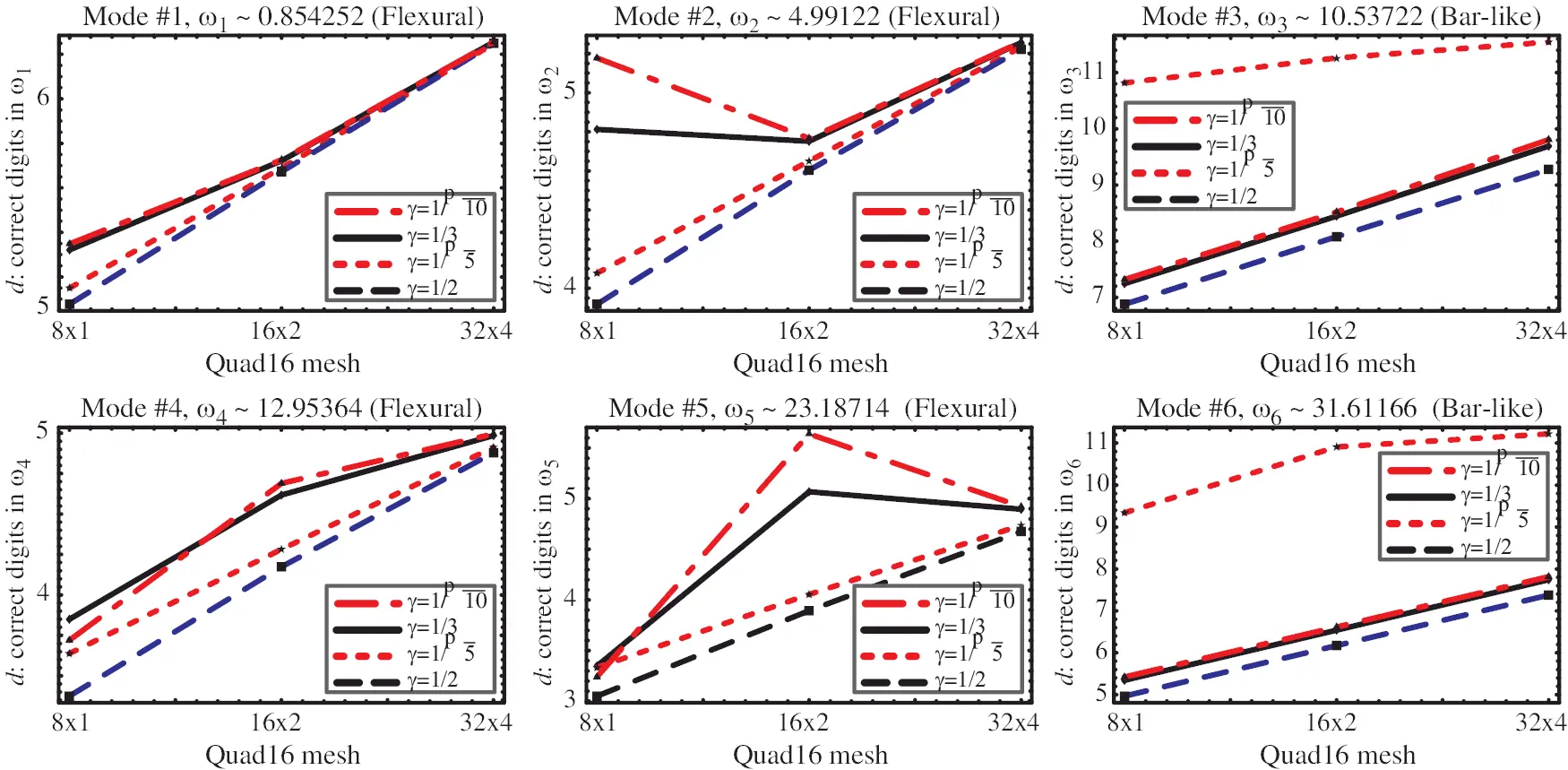Figure 15: Accuracy of computed Quad16 frequencies for three meshes (8 × 1 through 32 × 4) with μ = 3/4, as affected by parameter γ

Three comments are in order for the Quad16 results. First, the flexural-mode convergence lines in Fig. 15 appear more “spread out” than those in Figs. 13 and 14. The reason is that the d scale is compressed on account of the high accuracy provided by the coarsest 8 × 1 mesh, which yields 5 to 3 exact digits for the first 4 flexural modes (Note that Quad4 gives less than one). Second, the jagged appearance of some plots is due to nonmonotonicity (only μ = 0 would guarantee monotonic convergence). Third, the ωi accuracy of the eigensolver levels out at approximately 12 digits, which explains the incipient “flattening” of the bar-like modes.

10  Conclusions

The main results of this paper are summarized next.

•   Presents the first detailed study, using plane wave packets as test functions, of the effect of adjusting side node locations of Lagrangian cubic finite elements on low-frequency accuracy.

•   The combined effect of reduced integration of the mass and/or stiffness matrix is considered.

•   For cubic bar elements, acoustic branch superconvergence is obtained by placing the side nodes at Lobatto points, and selecting a special combination of the mass and stiffness matrix. One remarkable result: 6 digits in ω1 with one element, see Table 10.

•   For Lagrangian cubic plane stress elements, superconvergence is retained for bar-like vibration modes. This behavior continues in regular 2D meshes for bar-like modes, and pressumably in 3D. But it disappears for modes that involve rotation, flexure and shear. This is not surprising since axial plane wave packets (P-waves) are plainly insufficient; S-waves should be also included as test functions in 2D and 3D Fourier analysis.

Whether superconvergent stiffness-mass combinations can be constructed for general 2D and 3D motions using the general theory of mass-stiffness templates  is an open question. Challenges associated with symbolic analysis in multiple dimensions are discussed in Appendix E of that paper.

Funding Statement: This paper expands on work conducted during the 2005--2006 summer academic recesses while the author was a visitor at CIMNE (Centro Internacional de Métodos Numéricos en Ingenieria) at Barcelona, Spain. The visits were partly supported by fellowships awarded by the Spanish Ministerio de Educación y Cultura during May-June of those years, and partly by the National Science Foundation under grant High-Fidelity Simulations for Heterogeneous Civil and Mechanical Systems, CMS-0219422. Special thanks are due to Professor Loc Vu-Quoc for organizing the Special CMES Issue in celebration of Karl Pister’s 95th birthday.

Conflicts of Interest: The author declares that he has no conflict of interest regarding the present study.

## References

1. Felippa, C. A. (2021). Remembrances of Berkeley in the Mid-Sixties.
2. Felippa, C. A. (1994). A survey of parametrized variational principles and applications to computational mechanics. Computer Methods in Applied Mechanics and Engineering, 113(1--2), 109-139. [Google Scholar] [CrossRef]
3. Atluri, S. N., Gallagher, R. N., Zienkiewicz, O. C. (1983). Hybrid and mixed finite element methods, Chichester and New York: Wiley-Interscience.
4. Pian, T. H. H., Wu, C. C. (2006). Hybrid and Incompatible Finite Element Methods. Boca Raton, FL: Chapman & Hall/CRC.
5. Wilson, E. L., Taylor, R. E., Doherty, W., Ghaboussi, J. (1973). Incompatible displacement models. In Numerical and Computer Methods in Structural Mechanics, pp. 43–57. New York: Academic Press.
6. Bergan, P. G., & Nygård, M. (1984). Finite elements with increased freedom in choosing shape functions. International Journal for Numerical Methods in Engineering, 20(4), 643-663. [Google Scholar] [CrossRef]
7. Hughes, T. J. R. (1987). The finite element method: Linear static and dynamic finite element analysis. Prentice Hall, Englewood Cliffs, NJ.
8. Felippa, C. A., & Oñate, E. (2021). Accurate timoshenko beam elements for linear elastostatics and LPB stability. Archives of Computational Methods in Engineering, 28(3), 2021-2080. [Google Scholar] [CrossRef]
9. Felippa, C. A., Guo, Q., & Park, K. C. (2015). Mass matrix templates: General description and 1D examples. Archives of Computational Methods in Engineering, 22(1), 1-65. [Google Scholar] [CrossRef]
10. Felippa, C. A. (2001). Customizing the mass and geometric stiffness of plane thin beam elements by Fourier methods. Engineering Computations, 18((1–2),), 286-303. [Google Scholar] [CrossRef]
11. Felippa, C. A. (2006). Construction of customized mass-stiffness pairs using templates. Journal of Aerospace Engineering, 19(4), 241-258. [Google Scholar] [CrossRef]
12. Felippa, C. A. (1966). Refined finite element analysis of linear and nonlinear two-dimensional structures (Ph.D. Dissertation). Department of Civil Engineering, University of California at Berkeley, Berkeley, CA.
13. Felippa, C. A., Clough, R. W. (1969). The finite element method in solid mechanics. In: Numerical Solution of Field Problems in Continuum Physics, SIAM–AMS Proceedings II, pp. 210–252. American Mathematical Society, Providence, RI.
14. Hinton, E., Rock, T., & Zienkiewicz, O. C. (1976). A note on mass lumping and related processes in the finite element method. Earthquake Engineering & Structural Dynamics, 4(1), 245-249. [Google Scholar] [CrossRef]
15. Olovsson, L., Simonsson, K., & Unosson, M. (2005). Selective mass scaling for explicit finite element analysis. International Journal for Numerical Methods in Engineering, 63(10), 1436-1445. [Google Scholar] [CrossRef]
16. Tkachuk, A., & Bischoff, M. (2013). Variational methods for selective mass scaling. Computational Mechanics, 52(3), 563-570. [Google Scholar] [CrossRef]
17. Udwadia, F. E., & Schutte, A. D. (2010). Equations of motion for general constrained systems in Lagrangian mechanics. Acta Mechanica, 213(1), 111-129. [Google Scholar] [CrossRef]
18. Tkachuk, A., Wolfmuth, B., & Bischoff, M. (2013). Hybrid-mixed discretization of elasto-dynamics contact problems using consistent singular mass matrices. International Journal for Numerical Methods in Engineering, 94(5), 473-493. [Google Scholar] [CrossRef]
19. Udwadia, F. E., & Phohomshiri, P. (2006). Explicit equations of motion for constrained mechanical systems with singular mass matrices and applications to multi-body dynamics. Proceedings of the Royal Society A: Mathematical, Physical and Engineering Sciences, 462(2071), 2097-2117. [Google Scholar] [CrossRef]
20. Abramowitz, N., Stegun, I. A. (1964). Handbook of mathematical functions with formulas, graphs, and mathematical tables. Applied Mathematics Series, vol. 55, National Bureau of Standards, U.S. Department of Commerce, Washington, D.C. Reprinted by Wiley, 1993.
21. MacNeal, R. H. (1970). The NASTRAN theoretical manual, vol. 221. Washington, D.C.: Scientific and Technical Information Office, National Aeronautics and Space Administration (NASA).
22. Belytschko, T., Mullen, R. (1978). On dispersive properties of finite element solutions. In: Modern problems in elastic wave propagation, pp. 67–82. New York: Wiley.
23. Wimp, J. (1981). Sequence transformations and their applications. New York: Academic Press.
24. Bergan, P. G., & Felippa, C. A. (1987). A triangular membrane element with rotational degrees of freedom. Computer Methods in Applied Mechanics and Engineering, 50(1), 25-69. [Google Scholar] [CrossRef]
25. Felippa, C. A., & Bergan, P. G. (1987). A triangular plate bending element based on an energy-orthogonal free formulation. Computer Methods in Applied Mechanics and Engineering, 61(2), 129-160. [Google Scholar] [CrossRef]
26. Bergan, P. G., & Hanssen, L. (1976). A new approach for deriving ‘good’ finite elements. The mathematics of finite elements and applications, 2, 483-498. [Google Scholar]
27. Bergan, P. G. (1980). Finite elements based on energy-orthogonal functions. International Journal for Numerical Methods in Engineering, 15(10), 1541-1555. [Google Scholar] [CrossRef]
28. Militello, C., & Felippa, C. A. (1990). A variational justification of the assumed natural strain formulation of finite elements: I. Variational principles. Computers & Structures, 34(3), 431-438. [Google Scholar] [CrossRef]
29. Militello, C., & Felippa, C. A. (1990). A variational justification of the assumed natural strain formulation of finite elements: II. The four-node plate element. Computers & Structures, 34(3), 439-444. [Google Scholar] [CrossRef]
30. Militello, C., & Felippa, C. A. (1991). The first ANDES elements: 9-DOF plate bending triangles. Computer Methods in Applied Mechanics and Engineering, 93(2), 217-246. [Google Scholar] [CrossRef]
31. Felippa, C. A. (2004). A template tutorial. In: Computational mechanics: Theory and practice: A book dedicated to Professor P. G. Bergan, pp. 29–68. Barcelona, Spain: CIMNE Publications.
32. Felippa, C. A. (2000). Recent advances in finite element templates. In: Topping, B. H. V. (ed.), Computational mechanics for the Twenty-First Century, pp. 71–98. Edinburg, UK: Saxe-Coburn Publications.
33. Felippa, C. A. (2003). A study of optimal membrane triangles with drilling freedoms. Computer Methods in Applied Mechanics and Engineering, 192(16–18), 2125-2168. [Google Scholar] [CrossRef]
34. Felippa, C. A. (2006). Supernatural QUAD4: A template formulation. Computer Methods in Applied Mechanics and Engineering, 195(41–43), 5316-5342. [Google Scholar] [CrossRef]

Appendix A

The template approach to finite element construction gradually emerged from a series of serendipitous discoveries. The starting point was work of the author with Pål Bergan in 1982 while he was on a sabbatical at Stanford. The work focused on the development of high performance 3-node triangle elements for plane stress  and Kirchhoff plate bending  using the Free Formulation (FF) of Bergan and Nygård [6,26,27]. The elements were eventually combined to form a shell element and implemented into the corotational nonlinear program FENRIS used by Der Norske Veritas for analyzing marine structures under extreme conditions. The plane stress element was the first 3-node membrane triangle with drilling DOF that was rank sufficient and free of aspect ratio locking.

As the name implies, FF allows substantial freedom in element development. In particular conformity can be ignored; all that matters is satisfying the Individual Patch Test that can be tested on a single element. Ensuing developments showed that free parameters naturally appeared in the stiffness matrix—as well as the mass and geometric stiffness matrices when the formulation was extended to dynamic and stability analysis, respectively. Which values should be given? Obviously the decision cannot be left to the discretion of program users, who might have no idea of the background theory. They were assigned using various optimality criteria. Free parameters kept appearing in FF successors, such as the Assumed Natural Deviatoric Strain (ANDES) method [28,29,30].

From special cases a general approach eventually took shape. It was named the template method in a 1994 journal paper . A fuller historical account is provided in a tutorial chapter . The general concept of template as parametrized forms of FEM matrix equations is discussed in [10,11,32,33,34]. The main advantage of templates is flexibility. This brings the attendent ability to customize elements without worries about complying with restrictions such as interelement conformity. The approach can generate all possible convergent elements; the main question left is which one to pick up. This rosy picture, however, is clouded by practical difficulties:

•   Element development computations must be done symbolically, carrying along material, fabrication and geometric information as variables. Such manipulations lie beyond human powers, and require a computer algebra system (CAS).

•   Symbolic computational work effort grows exponentially in the number of free parameters as well as matrix order. In addition, symbolic eigenvalue analysis in stability and dynamic problems rapidly becomes difficult or impossible as the chacteristic polynomial order increases.

With current CAS the treatment of 1D elements is possible, as well as that of 2D elements of simple geometry. But 2D and 3D elements of arbitrary geometry (for instance, doubly curved shells) lie outside the scope of current computers and CAS power.This work is licensed under a Creative Commons Attribution 4.0 International License, which permits unrestricted use, distribution, and reproduction in any medium, provided the original work is properly cited.